×

# 6805 computer

8-bit FAST Microcontrollers Family
This 6805 SOC is an advanced 8-bit MCU IP Core with highly sophisticated, on chip peripheral capabilities. The 6805 soft core is binary-compatible with the industry standard Motorola 68HC05 8-bit microcontroller and can achieve a performance 45-100 million instructions per second. This 6805 Microcontroller Core contains a mini UART, two 8-bit timer, read-only memory (ROM), DoCDTM(DCD Hardware Debug System) and can also be equipped with the Synchronous Serial Peripheral Interface (SPI). .

In contrast to other on-chip debuggers the DoCD provides a non-intrusive debugging of running application. It can halt, run, step into or skip an instruction, read/write any contents of microcontroller including all registers, SFRs including user defined peripherals, data and program memories.

Features:

• M68HC05 core
• Fully static operation
• 64 bytes of user ROM
• 131 Kbytes of on-chip random-access memory (RAM)
• Mini uart
• Two 8-bit timer system
• 32 bidirectional input/output (I/O) lines
• Software compatible with industry standard 68HC05
• 11 times faster multiplication
• Upto 32 Mbytes of System Function Registers space (SFRs)
• 8 bytes of BSET rom
• 8K bytes of BCLR rom
• Diagnostic LED Flasher
• Fully synthesizable
• 25 MHz of virtual clock frequency compared to original implementation
Note: A line over a signal name indicates an active low signal.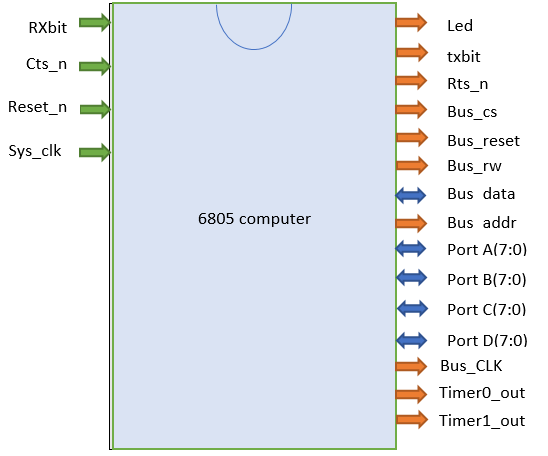Pin Description

PIN Type Interface Description
Rxbit Input Mini-Uart RS-232 data input
Txbit Output Mini-Uart RS-232 data output
RTS_n Output Mini-Uart Request To Send (Active low)
CTS_n Input Mini-Uart Clear To Send (Active low)
Bus_DATA inout External Bus Bus Data
Bus_RW Output External Bus Bus READ/WRITE
Bus_CS Output External Bus Bus Chip select
Bus_CLK Output External Bus Clock
Timer_0 Output timer 8 bit timer
Timer_1 Output timer 8 bit timer
Sys_clk Input control unit System Clock input
Reset_nInputcontrol unit This active low pin is used to reset the MCU to a known startup state by pulling RESET low.
LED Output control unit Diagnostic LED Flasher
Bus_RESET Output External Bus Reset external bus
portA (7:0)Inout I/O port 8 software programmable I/O
portB (7:0)Inout I/O port 8 software programmable I/O
portC (7:0)Inout I/O port 8 software programmable I/O
portC (7:0)Inout I/O port 8 software programmable I/O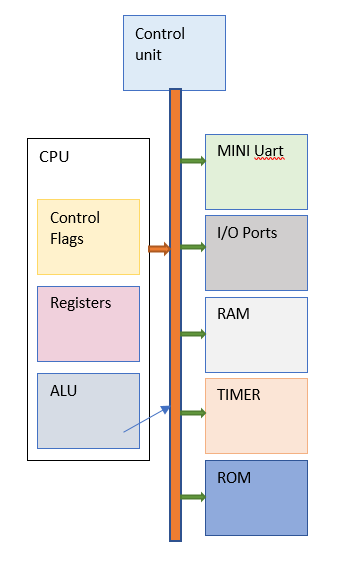We will discuss each block as a separate topic including their Verilog code, simulation, testing and working .

• 1. Cpu -Consists of the ALU, Registers and Control flags.
• 2. Control unit - Controls flow of signal between the cpu and the rest of the computer.
• 3. Mini-UART - Used for sending Signal TO and FRO using RS-232 protocol.
• 4. I/O Ports – Input/output ports for receiving and sending signals.
• 5. RAM - Memory storage .
• 6. Timer - it consist of 2 X 8 Bit timer.
• 7. Boot Rom - Used on computer start-up

### 1. TIMER:

The computer has two 8-bit, software-programmable counter. the `timer0 ` is driven by a fixed divide-by-sixteen prescaler while `timer1` is driven by a divide-by-sixty four prescaler . This timer can be used for many purposes, including input waveform measurements while simultaneously generating an output waveform. Pulse widths can vary from several microseconds to many seconds. The timer is set by turning on the timer chip select from the control unit, and updating the counter using data from the CPU. CPU_address` 2:0` is used to select between `timer1`, `timer0` and the timer control register. A value of zero selects the timer control register , a value of 2 selects `timer1` and a value of 3 selects `timer0`. The key element in the programmable timer is a 24-bit, free-running counter or counter register, preceded by a prescaler that divides the internal processor clock by either 16 or 64 for `timer0` and `timer1` respectively. The counter is incremented during the low portion of the internal bus clock for timer0 and during the high portion of the internal bus clock for timer1. This gives the timer a resolution of 0.64nano seconds and 2.56nano seconds respectively. The operating system clock if assumed to be 25Mhz., The timer clocks will be operating at :

Timer0 = 25,000 / 16= 1.562 MHz
Timer1 = 25,000 / 64 = 390 Khz
So, assume you want a pulse every 100 microseconds, with 25MHz system clock .then:
1/10-4 = 0.0001secs
1/0.0001 = 10,000Hz = 10KHz
(1.562 X 10^6)/(10^4) = 1.562 X 10^2 = 156.2 =approx.. 1001 1100
So the timer data_in will be fed with 10011100, with cs bit enables ,
While RW will be disabled(i.e Write mode) and the address bit should be 010 for timer0.
So a different calculation should be made for timer1.

Pin description

Pin Port Interface Description
Data_out (7:0)OutputControl unitRegister of the selected timer input
Tim0_out Output External Peripheral . Output of timer1
Tim1_out Output External Peripheral . Output of timer2.
IRQ_OUT Output CPU. Interrupt request which is bit1 of `cpu_data_in` when CPU address selected is timer control register .
Tim0_in Input Control unit Timer0 input
Tim1_in Input Control unit Timer1 input
Reset Input cpu_reset Reset
Clock Input cpu_clk clock
R/W Input CPU Read / Not Write
Timer_CS Input Control unit indicates the chip selected for cpu data is the timer.
Data_in(7:0) Input CPU Data Input from the CPU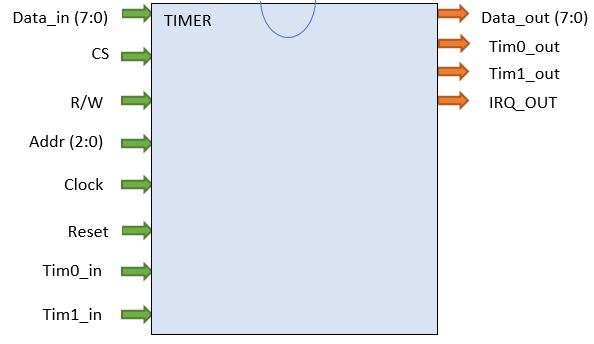```library ieee;
use ieee.std_logic_1164.all;
use ieee.std_logic_unsigned.all;

entity timer is
port (
clk       : in  std_logic;
rst       : in  std_logic;
cs        : in  std_logic;
rw        : in  std_logic;
addr      : in  std_logic_vector(2 downto 0);
data_in   : in  std_logic_vector(7 downto 0);
data_out  : out std_logic_vector(7 downto 0);
irq_out   : out std_logic;
tim0_in   : in  std_logic;
tim0_out  : out std_logic;
tim1_in   : in  std_logic;
tim1_out  : out std_logic
);
end;

architecture timer_arch of timer is
signal timer_ctrl_reg : std_logic_vector(7 downto 0);
signal timer0_reg : std_logic_vector(7 downto 0);
signal timer1_reg : std_logic_vector(7 downto 0);
signal count0     : std_logic_vector(7 downto 0);
signal count1     : std_logic_vector(7 downto 0);
begin

--------------------------------
--
-- write control registers
-- doesn't do anything yet
--
--------------------------------
write_timer_control : process( clk, rst, cs, rw, addr, data_in, timer0_reg, timer1_reg, timer_ctrl_reg )
begin
if clk'event and clk = '0' then
if cs = '1' and rw = '0' then
when "000" =>
timer_ctrl_reg <= data_in;
timer0_reg <= timer0_reg;
timer1_reg <= timer1_reg;
when "010" =>
timer_ctrl_reg <= timer_ctrl_reg;
timer0_reg <= data_in;
timer1_reg <= timer1_reg;
when "011" =>
timer_ctrl_reg <= timer_ctrl_reg;
timer0_reg <= timer0_reg;
timer1_reg <= data_in;
when others =>
timer_ctrl_reg <= timer_ctrl_reg;
timer0_reg <= timer0_reg;
timer1_reg <= timer1_reg;
end case;
else
timer_ctrl_reg <= timer_ctrl_reg;
timer0_reg <= timer0_reg;
timer1_reg <= timer1_reg;
end if;
end if;
end process;

begin
when "000" =>
data_out <= timer_ctrl_reg;
when "010" =>
data_out <= timer0_reg;
when "011" =>
data_out <= timer1_reg;
when "110" =>
data_out <= count0;
when "111" =>
data_out <= count1;
when others =>
data_out <= "00000000";
end case;
irq_out <= timer_ctrl_reg(0);
end process;

--------------------------------
--
-- counters
--
--------------------------------

my_counter: process( clk, rst, count0, count1, tim0_in, tim1_in )
begin
if rst = '1' then
count0 <= "00000000";
elsif tim0_in'event and tim0_in = '0' then
if count0 = timer0_reg then
count0 <= "00000000";
else
count0 <= count0 + 1;
end if;
end if;

if rst = '1' then
count1 <= "00000000";
elsif tim1_in'event and tim1_in = '1' then
if count1 = timer1_reg then
count1 <= "00000000";
else
count1 <= count1 + 1;
end if;
end if;

tim0_out <= count0(7);
tim1_out <= count1(7);
end process;

end timer_arch;

```

### 2. MINI-UART:

The Mini-UART is an interface built into the 6805soc which allows several communication between the SOC and any RS-232 enabled device example your PC.It is capable of sending parallel data through a serial line.

Features:

• Speed: standard 58 KBaud Clock.
• Full duplex, transmission and reception can be carried out simultaneously
• Error checking capabilities: frame timing checks, parity error checks, output error
• Programmable bit rate
• Programmable word format including “N81” Format.
• Transmit / Receive with h/w handshake
• No internal Fifo (usually not needed in an FPGA!)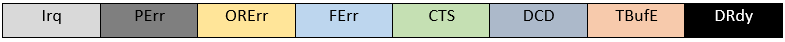The UART has a 8-bit status register that keeps record of Rx Status:

Bit description

• TBufE → Transmit buffer empty
• DCD → Data Carrier Detect
• CTS → Clear to send
• FErr → Framing error
• ORErr → Overrun error
• PErr → Parity Error
• IRQ → Interrupt Request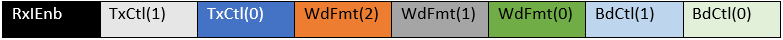The UART Control Register has 8 – bits indicating options for bit size, word format, Wether inclusion of parity or no parity.

`RxIEnb` bit

• 0 → Rx Interrupt disabled
• 1 →Rx Interrupt enabled

` TxCtl` bit

• 0 1→ Tx Interrupt Enable
• 1 0 → RTS high

`WdFmt` bit => this bits is used for the configuration of the size and arrangement of bits to be transferred or received.
• 0 0 0 → 7 data, even parity, 2 stop
• 0 0 1 → 7 data, odd parity, 2 stop
• 0 1 0 → 7 data, even parity, 1 stop
• 0 1 1 → 7 data, odd parity, 1 stop
• 1 0 0 → 8 data, no parity, 2 stop
• 1 0 1 → 8 data, no parity, 1 stop
• 1 1 0 → 8 data, even parity, 1 stop
• 1 1 1 → 8 data, odd parity, 1 stop

The UART consist of a receiver and a transmitter, which share a baud clock of 58 KBaud with ` BdCtl` bit of 01 or 14KBaud for `BdCtl` bit of 10. With our 25MHz System clock, the UART clock counter has a divide by 21 counter .which divides the system clock by :

25,000,000 / 27 = 926,000 KBaud when `BdCtl` bit is 00.
926,000 / 16 = 58 KBaud when ` BdCtl `bit is 01.
Baud Rate Generator Clock Prescaling

`BdCtl` bit

• 0 0 → Baud Clk divide by 1
• 0 1 → Baud Clk divide by 16
• 1 0 → Baud Clk divide by 64
• 1 1 → reset

Pin Description

Pin Type Port Description
Reset. Input cpu_reset Reset input (active high)
Clock. Input cpu_clk System Clock
CS. Input control unit miniUART Chip Select
RW. Input CPU Read / Not Write
IRQ. Output CPU Interrupt
Data_in. Input CPU Data Bus In
Data_out. Input Control unit Data Bus Out
RxC. Output Control unit Receive Baud Clock
TxC. Input Control unit Transmit Baud Clock
TxD. Output RS-232 Transmit Data
DCD_n. Input Control Unit Data Carrier Detect (Active low)
CTS_n. Input RS-232 Clear To Send (Active low)
RTS_n. Output RS-232 Request To Send (Active low)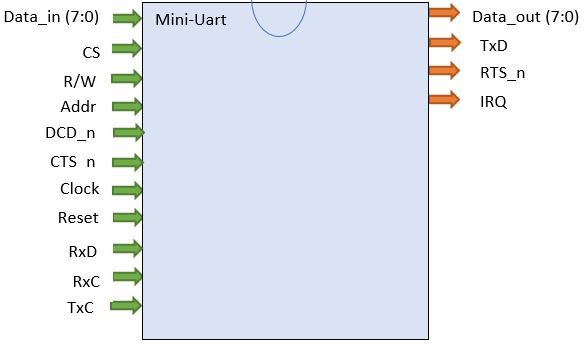```library ieee;
use ieee.std_logic_1164.all;
use ieee.std_logic_unsigned.all;

-------------------------------------------------------------------------------
-------------------------------------------------------------------------------
entity RxUnit is
port (
Clk    : in  Std_Logic;  -- Clock signal
Reset  : in  Std_Logic;  -- Reset input
WdFmt  : in  Std_Logic_Vector(2 downto 0); -- word format
BdFmt  : in  Std_Logic_Vector(1 downto 0); -- baud format
RxClk  : in  Std_Logic;  -- RS-232 clock input
RxDat  : in  Std_Logic;  -- RS-232 data input
FRErr  : out Std_Logic;  -- Status signal
ORErr  : out Std_Logic;  -- Status signal
PAErr  : out Std_logic;  -- Status signal
DARdy  : out Std_Logic;  -- Status signal
DAOut  : out Std_Logic_Vector(7 downto 0)
);
end; --================== End of entity ==============================--
-------------------------------------------------------------------------------
-------------------------------------------------------------------------------
architecture Behaviour of RxUnit is
-----------------------------------------------------------------------------
-- Signals
-----------------------------------------------------------------------------
signal RxDebDel0  : Std_Logic;             -- Debounce Delayed Rx Data
signal RxDebDel1  : Std_Logic;             -- Debounce Delayed Rx Data
signal RxDebDel2  : Std_Logic;             -- Debounce Delayed Rx Data
signal RxDebDel3  : Std_Logic;             -- Debounce Delayed Rx Data
signal RxDeb      : Std_Logic;             -- Debounced Rx Data
signal RxDatDel   : Std_Logic;             -- Delayed Rx Data
signal RxDatEdge  : Std_Logic;             -- Rx Data Edge pulse
signal RxClkDel   : Std_Logic;             -- Delayed Rx Input Clock
signal RxClkEdge  : Std_Logic;             -- Rx Input Clock Edge pulse
signal RxClkCnt   : Std_Logic_Vector(5 downto 0); -- Rx Baud Clock Counter
signal RxBdClk    : Std_Logic;             -- Rx Baud Clock
signal RxBdDel    : Std_Logic;             -- Delayed Rx Baud Clock
signal RxBdEdge   : Std_Logic;             -- Rx Baud Clock Edge pulse
signal RxStart    : Std_Logic;					-- Rx Start bit detected

signal tmpDRdy    : Std_Logic;             -- Data Ready flag
signal RxValid    : Std_Logic;             -- Rx Data Valid
signal tmpRxVal   : Std_Logic;             -- Rx Data Valid
signal outErr     : Std_Logic;             -- Over run error bit
signal frameErr   : Std_Logic;             -- Framing error bit
signal ParityErr  : Std_Logic;             -- Parity Error Bit
signal RxParity   : Std_Logic;             -- Calculated RX parity bit
signal RxState    : Std_Logic_Vector(3 downto 0);  -- receive bit state
signal ShtReg     : Std_Logic_Vector(7 downto 0);  -- Shift Register
signal DataOut    : Std_Logic_Vector(7 downto 0);  -- Data Output register

begin

---------------------------------------------------------------------
-- Input level must be stable for 4 Receive Clock cycles.
---------------------------------------------------------------------
rxunit_data_debounce : process(Clk, Reset, RxClkEdge, RxDat,
RxDebDel0, RxDebDel1, RxDebDel2, RxDebDel3 )
begin
if Reset = '1' then
RxDebDel0 <= RxDat;
RxDebDel1 <= RxDat;
RxDebDel2 <= RxDat;
RxDebDel3 <= RxDat;
elsif Clk'event and Clk = '0' then
if RxClkEdge = '1' then
RxDebDel0  <= RxDat;
RxDebDel1  <= RxDebDel0;
RxDebDel2  <= RxDebDel1;
RxDebDel3  <= RxDebDel2;
if (RxDebDel3 or RxDebDel2 or RxDebDel1 or RxDebDel0) = '0' then
RxDeb <= '0';
elsif (RxDebDel3 and RxDebDel2 and RxDebDel1 and RxDebDel0) = '1' then
RxDeb <= '1';
else
RxDeb <= RxDeb;
end if;
else
RxDebDel0  <= RxDebDel0;
RxDebDel1  <= RxDebDel1;
RxDebDel2  <= RxDebDel2;
RxDebDel3  <= RxDebDel3;
RxDeb      <= RxDeb;
end if;
end if;
end process;

---------------------------------------------------------------------
-- A falling edge will produce a one clock cycle pulse
---------------------------------------------------------------------
rxunit_data_edge : process(Clk, Reset, RxDeb, RxDatDel )
begin
if Reset = '1' then
RxDatDel  <= RxDeb;
RxDatEdge <= '0';
elsif Clk'event and Clk = '0' then
RxDatDel  <= RxDeb;
RxDatEdge <= RxDatDel and (not RxDeb);
end if;
end process;

---------------------------------------------------------------------
-- A rising edge will produce a one clock cycle pulse
-- RxClock
---------------------------------------------------------------------
rxunit_clock_edge : process(Clk, Reset, RxClk, RxClkDel )
begin
if Reset = '1' then
RxClkDel  <= RxClk;
RxClkEdge <= '0';
elsif Clk'event and Clk = '0' then
RxClkDel  <= RxClk;
RxClkEdge <= RxClk and (not RxClkDel);
end if;
end process;

---------------------------------------------------------------------
-- Reset the Rx Clock divider on any data edge
-- Note that debounce data will be skewed by 4 clock cycles.
-- Advance the count only on an input clock pulse
---------------------------------------------------------------------
rxunit_clock_divide : process(Clk, Reset, RxDatEdge, RxState, RxStart,
RxClkEdge, RxClkCnt )
begin
if Reset = '1' then
RxClkCnt  <= "000000";
RxStart   <= '0';
elsif Clk'event and Clk = '0' then

if RxState = "1111" then     -- idle state
if RxStart = '0' then      -- in hunt mode
if RxDatEdge = '1' then -- falling edge starts counter
RxStart <= '1';
else
RxStart <= RxStart;	  -- other wise remain halted
end if;
else
RxStart <= RxStart;		  -- Acquired start, stay in this state
end if;
else
RxStart <= '0';	           -- non idle, reset start flag
end if; -- RxState

if RxState = "1111" and RxStart = '0' then
RxClkCnt <= "000011";  -- Reset to 3 to account for debounce skew
else
if RxClkEdge = '1' then
RxClkCnt <= RxClkCnt + "000001";
else
RxClkCnt <= RxClkCnt;
end if; -- RxClkEdge
end if; -- RxState
end if;	 -- clk / reset
end process;

---------------------------------------------------------------------
-- Select output then look for rising edge
---------------------------------------------------------------------
rxunit_clock_select : process(Clk, Reset, BdFmt, RxClk, RxClkCnt,
RxBdDel, RxBdEdge )
begin
-- BdFmt
-- 0 0     - Baud Clk divide by 1
-- 0 1     - Baud Clk divide by 16
-- 1 0     - Baud Clk divide by 64
-- 1 1     - reset
case BdFmt is
when "00" =>	  -- Div by 1
RxBdClk <= RxClk;
when "01" =>	  -- Div by 16
RxBdClk <= RxClkCnt(3);
when "10" =>	  -- Div by 64
RxBdClk <= RxClkCnt(5);
when others =>  -- reset
RxBdClk <= '0';
end case;

if Reset = '1' then
RxBdDel  <= RxBdClk;
RxBdEdge <= '0';
elsif Clk'event and Clk = '0' then
RxBdDel  <= RxBdClk;
RxBdEdge <= RxBdClk and (not RxBdDel);
end if;

end process;

---------------------------------------------------------------------
---------------------------------------------------------------------
rxunit_receive : process(Clk, Reset, RxState, RxBdEdge, RxDat )
begin
if Reset = '1' then
frameErr  <= '0';
outErr    <= '0';
parityErr <= '0';

ShtReg    <= "00000000";  -- Shift register
DataOut   <= "00000000";
RxParity  <= '0';         -- Parity bit
RxValid   <= '0';         -- Data RX data valid flag
RxState   <= "1111";
elsif Clk'event and Clk='0' then
if RxBdEdge = '1' then
case RxState is
when "0000" | "0001" | "0010" | "0011" |
"0100" | "0101" | "0110" => -- data bits 0 to 6
ShtReg    <= RxDat & ShtReg(7 downto 1);
RxParity  <= RxParity xor RxDat;
parityErr <= parityErr;
frameErr  <= frameErr;
outErr    <= outErr;
RxValid   <= '0';
DataOut   <= DataOut;
if RxState = "0110" then
if WdFmt(2) = '0' then
RxState <= "1000";          -- 7 data + parity
else
RxState <= "0111";          -- 8 data bits
end if; -- WdFmt(2)
else
RxState   <= RxState + "0001";
end if; -- RxState

when "0111" =>                 -- data bit 7
ShtReg    <= RxDat & ShtReg(7 downto 1);
RxParity  <= RxParity xor RxDat;
parityErr <= parityErr;
frameErr  <= frameErr;
outErr    <= outErr;
RxValid   <= '0';
DataOut   <= DataOut;
if WdFmt(1) = '1' then      -- parity bit ?
RxState <= "1000";         -- yes, go to parity
else
RxState <= "1001";         -- no, must be 2 stop bit bits
end if;

when "1000" =>                 -- parity bit
if WdFmt(2) = '0' then
ShtReg <= RxDat & ShtReg(7 downto 1); -- 7 data + parity
else
ShtReg <= ShtReg;          -- 8 data + parity
end if;
RxParity <= RxParity;
if WdFmt(0) = '0' then      -- parity polarity ?
if RxParity = RxDat then  -- check even parity
parityErr <= '1';
else
parityErr <= '0';
end if;
else
if RxParity = RxDat then  -- check for odd parity
parityErr <= '0';
else
parityErr <= '1';
end if;
end if;
frameErr  <= frameErr;
outErr    <= outErr;
RxValid   <= '0';
DataOut   <= DataOut;
RxState   <= "1001";

when "1001" =>                 -- stop bit (Only one required for RX)
ShtReg    <= ShtReg;
RxParity  <= RxParity;
parityErr <= parityErr;
if RxDat = '1' then         -- stop bit expected
frameErr <= '0';           -- yes, no framing error
else
frameErr <= '1';           -- no, framing error
end if;
if tmpDRdy = '1' then        -- Has previous data been read ?
outErr <= '1';             -- no, overrun error
else
outErr <= '0';             -- yes, no over run error
end if;
RxValid   <= '1';
DataOut   <= ShtReg;
RxState   <= "1111";

when others =>                 -- this is the idle state
ShtReg    <= ShtReg;
RxParity  <= RxParity;
parityErr <= parityErr;
frameErr  <= frameErr;
outErr    <= outErr;
RxValid   <= '0';
DataOut   <= DataOut;
if RxDat = '0' then  -- look for start request
RxState  <= "0000"; -- yes, read data
else
RxState <= "1111";    -- otherwise idle
end if;
end case; -- RxState
else  -- RxBdEdge
ShtReg    <= ShtReg;
RxParity  <= RxParity;
parityErr <= parityErr;
frameErr  <= frameErr;
outErr    <= outErr;
RxValid   <= RxValid;
DataOut   <= DataOut;
RxState   <= RxState;
end if; -- RxBdEdge
end if; -- clk / reset
end process;

---------------------------------------------------------------------
---------------------------------------------------------------------
begin
if Reset = '1' then
tmpDRdy   <= '0';
tmpRxVal  <= '0';
elsif Clk'event and Clk='0' then
tmpDRdy  <= '0';
tmpRxVal <= tmpRxVal;
else
if RxValid = '1' and tmpRxVal = '0' then
tmpDRdy  <= '1';
tmpRxVal <= '1';
else
-- Test for falling edge of RxValid.
tmpDRdy <= tmpDRdy;
if RxValid = '0' and tmpRxVal = '1' then
tmpRxVal  <= '0';
else
tmpRxVal  <= tmpRxVal;
end if;
end if; -- RxValid
end if; -- clk / reset
end process;

DARdy <= tmpDRdy;
DAOut <= DataOut;
FRErr <= frameErr;
ORErr <= outErr;
PAErr <= parityErr;

end Behaviour; --==================== End of architecture ====================--
```
```library ieee;
use ieee.std_logic_1164.all;
use ieee.numeric_std.all;
use ieee.std_logic_unsigned.all;

-------------------------------------------------------------------------------
-- Transmitter unit
-------------------------------------------------------------------------------
entity TxUnit is
port (
Clk    : in  Std_Logic;  -- Clock signal
Reset  : in  Std_Logic;  -- Reset input
DAIn   : in  Std_Logic_Vector(7 downto 0);
WdFmt  : in  Std_Logic_Vector(2 downto 0); -- word format
BdFmt  : in  Std_Logic_Vector(1 downto 0); -- baud format
TxClk  : in  Std_Logic;  -- Enable input
TxDat  : out Std_Logic;  -- RS-232 data output
TBE    : out Std_Logic );  -- Tx buffer empty
end; --================== End of entity ==============================
-------------------------------------------------------------------------------
-- Architecture for TxUnit
-------------------------------------------------------------------------------
architecture Behaviour of TxUnit is
type TxStateType is ( TxIdle_State, Start_State, Data_State, Parity_State, Stop_State );
-----------------------------------------------------------------------------
-- Signals
-----------------------------------------------------------------------------
signal TxClkDel  : Std_Logic;             -- Delayed Tx Input Clock
signal TxClkEdge : Std_Logic;             -- Tx Input Clock Edge pulse
signal TxClkCnt  : Std_Logic_Vector(5 downto 0); -- Tx Baud Clock Counter
signal TxBdDel   : Std_Logic;             -- Delayed Tx Baud Clock
signal TxBdEdge  : Std_Logic;             -- Tx Baud Clock Edge pulse
signal TxBdClk    : Std_Logic;            -- Tx Baud Clock

signal TBuff     : Std_Logic_Vector(7 downto 0); -- transmit buffer
signal TBufE     : Std_Logic;                    -- Transmit Buffer Empty

signal TReg      : Std_Logic_Vector(7 downto 0); -- transmit register
signal TxParity  : Std_logic;                    -- Parity Bit
signal DataCnt   : Std_Logic_Vector(3 downto 0); -- Data Bit Counter
signal TRegE     : Std_Logic;                    --  Transmit Register empty
signal TRegEDel  : Std_Logic;                    --  Transmit Register empty
signal TRegEEdge : Std_Logic;
signal TxState   : TxStateType;
signal TxDbit    : Std_Logic;
begin

---------------------------------------------------------------------
-- Transmit Clock Edge Detection
-- A falling edge will produce a one clock cycle pulse
---------------------------------------------------------------------
txunit_clock_edge : process(Clk, Reset, TxClk, TxClkDel )
begin
if Reset = '1' then
TxClkDel  <= TxClk;
TxClkEdge <= '0';
elsif Clk'event and Clk = '0' then
TxClkDel  <= TxClk;
TxClkEdge <= TxClkDel and (not TxClk);
end if;
end process;

---------------------------------------------------------------------
-- Transmit Clock Divider
-- Advance the count only on an input clock pulse
---------------------------------------------------------------------
txunit_clock_divide : process(Clk, Reset, TxClkEdge, TxClkCnt )
begin
if Reset = '1' then
TxClkCnt <= "000000";
elsif Clk'event and Clk = '0' then
if TxClkEdge = '1' then
TxClkCnt <= TxClkCnt + "000001";
else
TxClkCnt <= TxClkCnt;
end if; -- TxClkEdge
end if;	-- reset / clk
end process;

---------------------------------------------------------------------
-- Select output then look for rising edge
---------------------------------------------------------------------
txunit_clock_select : process(Clk, Reset, BdFmt, TxClk, TxClkCnt,
TxBdDel, TxBdEdge )
begin
-- BdFmt
-- 0 0     - Baud Clk divide by 1
-- 0 1     - Baud Clk divide by 16
-- 1 0     - Baud Clk divide by 64
-- 1 1     - reset
case BdFmt is
when "00" =>	  -- Div by 1
TxBdClk <= TxClk;
when "01" =>	  -- Div by 16
TxBdClk <= TxClkCnt(3);
when "10" =>	  -- Div by 64
TxBdClk <= TxClkCnt(5);
when others =>  -- reset
TxBdClk <= '0';
end case;

if Reset = '1' then
TxBdDel  <= TxBdClk;
TxBdEdge <= '0';
elsif Clk'event and Clk = '0' then
TxBdDel  <= TxBdClk;
TxBdEdge <= TxBdClk and (not TxBdDel);
end if;
end process;

---------------------------------------------------------------------
-- Transmit Buffer Empty Edge
-- generate a negative edge pulse
---------------------------------------------------------------------
txunit_busy : process(Clk, Reset, TRegE, TRegEDel )
begin
if Reset = '1' then
TRegEDel  <= '0';
TRegEEdge <= '0';
elsif Clk'event and Clk = '0' then
TRegEDel  <= TRegE;
TRegEEdge <= TregEDel and (not TRegE ); -- falling edge
end if;
end process;

---------------------------------------------------------------------
-- Transmitter activation process
---------------------------------------------------------------------
txunit_write : process(Clk, Reset, LoadD, DAIn, TBufE, TRegEEdge )
begin
if Reset = '1' then
TBufE   <= '1';
TBuff   <= "00000000";
elsif Clk'event and Clk = '0' then
TBuff <= DAIn;
TBufE <= '0';
else
TBuff <= TBuff;
if (TBufE = '0') and (TRegEEdge = '1') then
-- Once the transmitter is started
-- We can flag the buffer empty again.
TBufE <= '1';
else
TBufE <= TBufE;
end if;
end if;
end if; -- clk / reset
TBE <= TBufE;

end process;

-----------------------------------------------------------------------------
-- Implements the Tx unit
-----------------------------------------------------------------------------
txunit_transmit :  process(Reset, Clk, TxState, TxDbit, TBuff, TReg,
TxBdEdge, TxParity, DataCnt, WdFmt,
TBufE, TRegE )
begin
if Reset = '1' then
TxDbit   <= '1';
TReg     <= "00000000";
TxParity <= '0';
DataCnt  <= "0000";
TRegE    <= '1';
TxState  <= TxIdle_State;
elsif Clk'event and Clk = '0' then
if TxBdEdge = '1' then
case TxState is
when TxIdle_State =>  -- TxIdle_State (also 1st or 2nd Stop bit)
TxDbit     <= '1';
TReg       <= TBuff;
TxParity   <= '0';
DataCnt    <= "0000";
TRegE      <= '1';
if TBufE = '0' then
TxState <= Start_State;
else
TxState <= TxIdle_State;
end if;

when Start_State =>
TxDbit   <= '0';           -- Start bit
TReg     <= TReg;
TxParity <= '0';
if WdFmt(2) = '0' then
DataCnt <= "0110";       -- 7 data + parity
else
DataCnt <= "0111";       -- 8 data
end if;
TRegE    <= '0';
TxState  <= Data_State;

when Data_State =>
TxDbit   <= TReg(0);
TReg     <= '1' & TReg(7 downto 1);
TxParity <= TxParity xor TReg(0);
TRegE    <= '0';
DataCnt  <= DataCnt - "0001";
if DataCnt = "0000" then
if (WdFmt(2) = '1') and (WdFmt(1) = '0') then
if WdFmt(0) = '0' then         -- 8 data bits
TxState <= Stop_State;       -- 2 stops
else
TxState <= TxIdle_State;     -- 1 stop
end if;
else
TxState <= Parity_State;       -- parity
end if;
else
TxState  <= Data_State;
end if;

when Parity_State =>           -- 7/8 data + parity bit
if WdFmt(0) = '0' then
TxDbit <= not( TxParity );   -- even parity
else
TXDbit <= TxParity;          -- odd parity
end if;
Treg     <= Treg;
TxParity <= '0';
TRegE    <= '0';
DataCnt  <= "0000";
if WdFmt(1) = '0' then
TxState <= Stop_State; -- 2 stops
else
TxState <= TxIdle_State; -- 1 stop
end if;

when Stop_State => -- first stop bit
TxDbit     <= '1';           -- 2 stop bits
Treg       <= Treg;
TxParity   <= '0';
DataCnt    <= "0000";
TRegE      <= '0';
TxState    <= TxIdle_State;

when others =>  -- Undefined
TxDbit     <= TxDbit;
Treg       <= Treg;
TxParity   <= '0';
DataCnt    <= "0000";
TRegE      <= TregE;
TxState    <= TxIdle_State;

end case; -- TxState

else -- TxBdEdge
TxDbit   <= TxDbit;
TReg     <= TReg;
TxParity <= TxParity;
DataCnt  <= DataCnt;
TRegE    <= TRegE;
TxState  <= TxState;
end if; -- TxBdEdge
end if;	 -- clk / reset

TxDat <= TxDbit;
end process;

end Behaviour; --=================== End of architecture ====================--
```
```library ieee;
use ieee.std_logic_1164.all;
use ieee.numeric_std.all;

entity miniUART is
port (
--
-- CPU signals
--
clk      : in  Std_Logic;  -- System Clock
rst      : in  Std_Logic;  -- Reset input (active high)
cs       : in  Std_Logic;  -- miniUART Chip Select
rw       : in  Std_Logic;  -- Read / Not Write
irq      : out Std_Logic;  -- Interrupt
Addr     : in  Std_Logic;  -- Register Select
DataIn   : in  Std_Logic_Vector(7 downto 0); -- Data Bus In
DataOut  : out Std_Logic_Vector(7 downto 0); -- Data Bus Out
--
-- Uart Signals
--
RxC      : in  Std_Logic;  -- Receive Baud Clock
TxC      : in  Std_Logic;  -- Transmit Baud Clock
RxD      : in  Std_Logic;  -- Receive Data
TxD      : out Std_Logic;  -- Transmit Data
DCD_n    : in  Std_Logic;  -- Data Carrier Detect
CTS_n    : in  Std_Logic;  -- Clear To Send
RTS_n    : out Std_Logic );  -- Request To send
end; --================== End of entity ==============================--
-------------------------------------------------------------------------------
-- Architecture for miniUART Controller Unit
-------------------------------------------------------------------------------
architecture uart of miniUART is
-----------------------------------------------------------------------------
-- Signals
-----------------------------------------------------------------------------
signal RxData : Std_Logic_Vector(7 downto 0); --
signal TxData : Std_Logic_Vector(7 downto 0); --
signal StatReg : Std_Logic_Vector(7 downto 0); -- status register
--             StatReg detailed
-----------+--------+--------+--------+--------+--------+--------+--------+
--  Irq    | PErr   | ORErr  | FErr   | CTS    | DCD    | TBufE  | DRdy   |
-----------+--------+--------+--------+--------+--------+--------+--------+
signal CtrlReg : Std_Logic_Vector(7 downto 0); -- control register
--             CtrlReg detailed
-----------+--------+--------+--------+--------+--------+--------+--------+
--  RxIEnb |TxCtl(1)|TxCtl(0)|WdFmt(2)|WdFmt(1)|WdFmt(0)|BdCtl(1)|BdCtl(0)|
-----------+--------+--------+--------+--------+--------+--------+--------+
-- RxIEnb
-- 0       - Rx Interrupt disabled
-- 1       - Rx Interrupt enabled
-- TxCtl
-- 0 1     - Tx Interrupt Enable
-- 1 0     - RTS high
-- WdFmt
-- 0 0 0   - 7 data, even parity, 2 stop
-- 0 0 1   - 7 data, odd  parity, 2 stop
-- 0 1 0   - 7 data, even parity, 1 stop
-- 0 1 1   - 7 data, odd  parity, 1 stop
-- 1 0 0   - 8 data, no   parity, 2 stop
-- 1 0 1   - 8 data, no   parity, 1 stop
-- 1 1 0   - 8 data, even parity, 1 stop
-- 1 1 1   - 8 data, odd  parity, 1 stop
-- BdCtl
-- 0 0     - Baud Clk divide by 1
-- 0 1     - Baud Clk divide by 16
-- 1 0     - Baud Clk divide by 64
-- 1 1     - reset

signal TxDbit   : Std_Logic;  -- Transmit data bit
signal TBufE    : Std_Logic;  -- Transmit buffer empty
signal FErr     : Std_Logic;  -- Frame error
signal OErr     : Std_Logic;  -- Output error
signal PErr     : Std_Logic;  -- Parity Error
signal TxIEnb   : Std_Logic;  -- Transmit interrupt enable
signal Reset    : Std_Logic;  -- Reset (Software & Hardware)
signal RxRst    : Std_Logic;  -- Receive Reset (Software & Hardware)
signal TxRst    : Std_Logic;  -- Transmit Reset (Software & Hardware)
signal DCDDel   : Std_Logic;  -- Delayed DCD_n
signal DCDEdge  : Std_Logic;  -- Rising DCD_N Edge Pulse
signal DCDState : Std_Logic;  -- DCD Reset sequencer
signal DCDInt   : Std_Logic;  -- DCD Interrupt

-----------------------------------------------------------------------------
-----------------------------------------------------------------------------
component RxUnit
port (
Clk     : in  Std_Logic;  -- Clock signal
Reset   : in  Std_Logic;  -- Reset input
WdFmt   : in  Std_Logic_Vector(2 downto 0); -- word format
BdFmt   : in  Std_Logic_Vector(1 downto 0); -- baud format
RxClk   : in  Std_Logic;  -- RS-232 clock input
RxDat   : in  Std_Logic;  -- RS-232 data input
FRErr   : out Std_Logic;  -- Status signal
ORErr   : out Std_Logic;  -- Status signal
PAErr   : out Std_logic;  -- Status signal
DARdy   : out Std_Logic;  -- Status signal
DAOut   : out Std_Logic_Vector(7 downto 0));
end component;
-----------------------------------------------------------------------------
-- Transmitter Unit
-----------------------------------------------------------------------------
component TxUnit
port (
Clk    : in  Std_Logic;  -- Clock signal
Reset  : in  Std_Logic;  -- Reset input
DAIn   : in  Std_Logic_Vector(7 downto 0);
WdFmt  : in  Std_Logic_Vector(2 downto 0); -- word format
BdFmt  : in  Std_Logic_Vector(1 downto 0); -- baud format
TxClk  : in  Std_Logic;  -- Enable input
TxDat  : out Std_Logic;  -- RS-232 data output
TBE    : out Std_Logic );  -- Tx buffer empty
end component;
begin
-----------------------------------------------------------------------------
-- Instantiation of internal components
-----------------------------------------------------------------------------

RxDev   : RxUnit  port map (
Clk      => clk,
Reset    => RxRst,
WdFmt    => CtrlReg(4 downto 2),
BdFmt    => CtrlReg(1 downto 0),
RxClk    => RxC,
RxDat    => RxD,
FRErr    => FErr,
ORErr    => OErr,
PAErr    => PErr,
DARdy    => DRdy,
DAOut    => RxData
);

TxDev   : TxUnit  port map (
Clk      => clk,
Reset    => TxRst,
DAIn     => TxData,
WdFmt    => CtrlReg(4 downto 2),
BdFmt    => CtrlReg(1 downto 0),
TxClk    => TxC,
TxDat    => TxDbit,
TBE      => TBufE
);

-----------------------------------------------------------------------------
-- Implements the controller for Rx&Tx units
-----------------------------------------------------------------------------
miniUart_Status : process(clk, Reset, CtrlReg, TxIEnb,
DRdy, TBufE, DCD_n, CTS_n, DCDInt,
FErr, OErr,  PErr )
variable Int : Std_Logic;
begin
if Reset = '1' then
Int     := '0';
StatReg <= "00000000";
irq     <= '0';
elsif clk'event and clk='0' then
Int        := (CtrlReg(7) and DRdy)   or
(CtrlReg(7) and DCDInt) or
(TxIEnb     and TBufE);
StatReg(1) <= TBufE and (not CTS_n); -- Transmit Buffer Empty
StatReg(2) <= DCDInt; -- Data Carrier Detect
StatReg(3) <= CTS_n; -- Clear To Send
StatReg(4) <= FErr;  -- Framing error
StatReg(5) <= OErr;  -- Overrun error
StatReg(6) <= PErr;  -- Parity error
StatReg(7) <= Int;
irq        <= Int;
end if;
end process;

-----------------------------------------------------------------------------
-- Transmit control
-----------------------------------------------------------------------------

miniUart_TxControl : process( CtrlReg, TxDbit )
begin
case CtrlReg(6 downto 5) is
when "00" => -- Disable TX Interrupts, Assert RTS
RTS_n  <= '0';
TxIEnb <= '0';
TxD    <= TxDbit;
when "01" => -- Enable TX interrupts, Assert RTS
RTS_n  <= '0';
TxIEnb <= '1';
TxD    <= TxDbit;
when "10" => -- Disable Tx Interrupts, Clear RTS
RTS_n  <= '1';
TxIEnb <= '0';
TxD    <= TxDbit;
when "11" => -- Disable Tx interrupts, Assert RTS, send break
RTS_n  <= '0';
TxIEnb <= '0';
TxD    <= '0';
when others =>
RTS_n  <= '0';
TxIEnb <= '0';
TxD    <= TxDbit;
end case;
end process;

-----------------------------------------------------------------------------
-- Write to control register
-----------------------------------------------------------------------------

miniUart_Control:  process(clk, Reset, cs, rw, Addr, DataIn, CtrlReg, TxData )
begin
if (reset = '1') then
TxData  <= "00000000";
CtrlReg <= "00000000";
elsif clk'event and clk='0' then
if cs = '1' then
if Addr = '1' then	-- Data Register
if rw = '0' then   -- write data register
TxData <= DataIn;
TxData <= TxData;
end if; -- rw
CtrlReg <= CtrlReg;
else					  -- Control / Status register
TxData <= TxData;
if rw = '0' then   -- write control register
CtrlReg <= DataIn;
CtrlReg <= CtrlReg;
end if; -- rw
else                   -- not selected
TxData  <= TxData;
CtrlReg <= CtrlReg;

end if;  -- cs
end if; -- clk / reset
end process;

---------------------------------------------------------------
--
-- set data output mux
--
--------------------------------------------------------------

begin
DataOut <= RxData;    -- read data register
else
DataOut <= StatReg;   -- read status register
end process;

---------------------------------------------------------------
--
-- Data Carrier Detect Edge rising edge detect
--
---------------------------------------------------------------
miniUart_DCD_edge : process( reset, clk, DCD_n, DCDDel	)
begin
if reset = '1' then
DCDEdge <= '0';
DCDDel  <= '0';
elsif clk'event and clk = '0' then
DCDDel <= DCD_n;
DCDEdge <= DCD_n and (not DCDDel);
end if;
end process;

---------------------------------------------------------------
--
-- Data Carrier Detect Interrupt
--
---------------------------------------------------------------
begin
if reset = '1' then
DCDInt   <= '0';
DCDState <= '0';
elsif clk'event and clk = '0' then
if DCDEdge = '1' then
DCDInt   <= '1';
DCDState <= '0';
elsif DCDState	= '0' then
-- To reset DCD interrupt, First read status
if (ReadCS <= '1') and (DCDInt = '1') then
DCDState <= '1';
else
DCDState <= '0';
end if;
DCDInt <= DCDInt;
else	-- DCDstate = '1'
-- Then read the data register
DCDState <= '0';
DCDInt <= '0';
else
DCDState <= DCDState;
DCDInt   <= DCDInt;
end if;
end if; -- DCDState
end if; -- clk / reset
end process;

---------------------------------------------------------------
--
-- reset may be hardware or software
--
---------------------------------------------------------------

miniUart_reset: process(rst, CtrlReg, Reset, DCD_n )
begin
Reset <= (CtrlReg(1) and CtrlReg(0)) or rst;
TxRst <= Reset;
RxRst <= Reset or DCD_n;
end process;

end; --===================== End of architecture =======================--
```

### 3. I/O PORTS:

The 6805soc has four 8-bit input/output (I/O) ports.These 32 port pins are programmable as either inputs or outputs under software control of the data direction registers and they do not share any of their pins with other subsystems. To avoid a glitch on the output pins, write data to the I/O port data register before writing a 1 to the corresponding data direction register.

Port A Port A is an 8-bit bidirectional port .The port A data register is at address `000` and the data direction register (DDR) is at address `100` of the CPU address. Reset sets the data register to zero , but clears the data direction registers, thereby returning the ports to inputs. Writing a 1 to a DDR bit sets the corresponding port bit to output mode.

Port B Port B is an 8-bit bidirectional port .The port B data register is at address `001` and the data direction register (DDR) is at address `101` of the CPU address. Reset sets the data register to zero , but clears the data direction registers, thereby returning the ports to inputs. Writing a 1 to a DDR bit sets the corresponding port bit to output mode.

Port C Port C is an 8-bit bidirectional port .The port C data register is at address `010` and the data direction register (DDR) is at address `110` of the CPU address. Reset sets the data register to zero , but clears the data direction registers, thereby returning the ports to inputs. Writing a 1 to a DDR bit sets the corresponding port bit to output mode.

Port D Port D is an 8-bit bidirectional port .The port D data register is at address `011` and the data direction register (DDR) is at address `111` of the CPU address. Reset sets the data register to zero , but clears the data direction registers, thereby returning the ports to inputs. Writing a 1 to a DDR bit sets the corresponding port bit to output mode.

I/O port pins may be programmed as inputs or outputs under software control. The direction of the pins is determined by the state of the corresponding bit in the port data direction register (DDR). Each I/O port has an associated DDR. Any I/O port pin is configured as an output if its corresponding DDR bit is set to a logic 1. A pin is configured as an input if its corresponding DDR bit is cleared to a logic 0.

At power-on or reset, all DDRs are cleared, which configures all I/O pins as inputs. The data direction registers are capable of being written to or read by the processor. During the programmed output state, a read of the data register actually reads the value of the output data latch and not the I/O pin.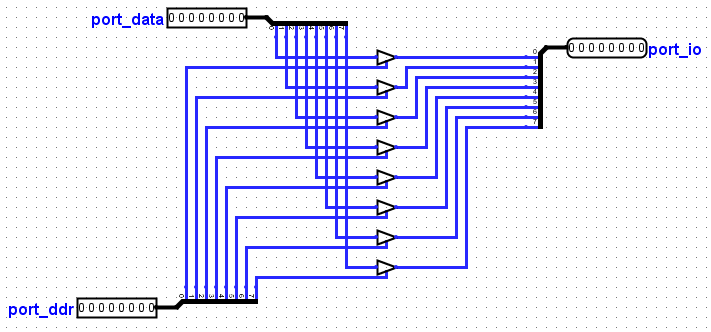The diagram above shows, how the Port data direction register controls the I/O function of the port using a controlled buffer. See that the port individual bits can be manipulated as input or output rather the whole port at once. Sending a 1 on any of the `Port_ddr` turns the port_data bit , thereby setting it as an output to the port_io.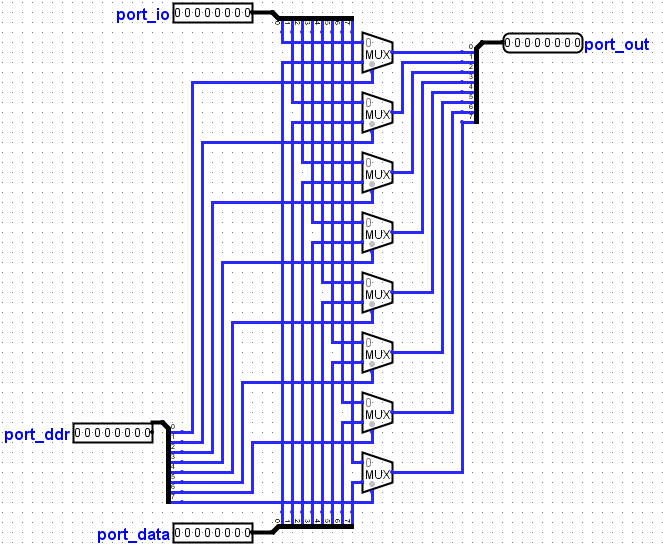The diagram above show how the port_ddr selects between input data(port_io) and output data(port_data) as a valid port data(port_out). Selecting a value of 1’ sets the selected multiplexer to allow the `port_data` bit which is the output data. When the `port_ddr` is set to zero, the specific multiplexer allows the `port_io` which is the input data.

Pin Description

PinType Interface Description
Reset Input cpu_reset Reset input (active high)
Clock Input cpu_clk System Clock
CS Input control unit I/O Chip Select
RW Input CPU Read / Not Write
ADDR(2:0) Input CPU Reset input (active high)
Data_in Input CPU Data In
Data_out Output control unit Data Out
PortA Inout External Peripheral PortA Bidirectional I/O
PortB Inout External Peripheral PortB Bidirectional I/O
PortC Inout External Peripheral PortC Bidirectional I/O
PortD Inout External Peripheral PortD Bidirectional I/O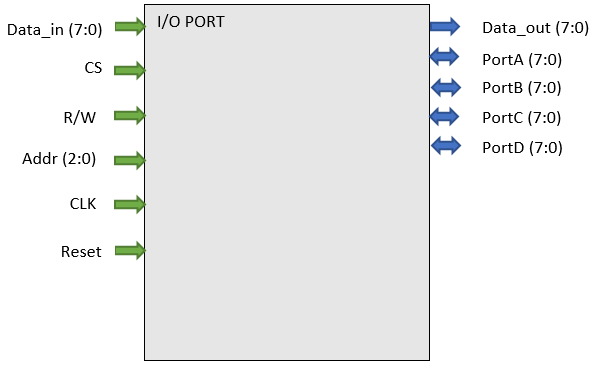```library ieee;
use ieee.std_logic_1164.all;
use ieee.std_logic_unsigned.all;

entity ioport is
port (
clk       : in  std_logic;
rst       : in  std_logic;
cs        : in  std_logic;
rw        : in  std_logic;
addr      : in  std_logic_vector(2 downto 0);
data_in   : in  std_logic_vector(7 downto 0);
data_out  : out std_logic_vector(7 downto 0);
porta_io  : inout std_logic_vector(7 downto 0);
portb_io  : inout std_logic_vector(7 downto 0);
portc_io  : inout std_logic_vector(7 downto 0);
portd_io  : inout std_logic_vector(7 downto 0) );
end;

architecture ioport_arch of ioport is
signal porta_ddr : std_logic_vector(7 downto 0);
signal portb_ddr : std_logic_vector(7 downto 0);
signal portc_ddr : std_logic_vector(7 downto 0);
signal portd_ddr : std_logic_vector(7 downto 0);
signal porta_data : std_logic_vector(7 downto 0);
signal portb_data : std_logic_vector(7 downto 0);
signal portc_data : std_logic_vector(7 downto 0);
signal portd_data : std_logic_vector(7 downto 0);

begin

--------------------------------
--
--
--------------------------------

porta_ddr, portb_ddr, portc_ddr, portd_ddr,
porta_data, portb_data, portc_data, portd_data,
porta_io, portb_io, portc_io, portd_io )
variable count : integer;
begin

when "000" =>
for count in 0 to 7 loop
if porta_ddr(count) = '1' then
data_out(count) <= porta_data(count);
else
data_out(count) <= porta_io(count);
end if;
end loop;

when "001" =>
for count in 0 to 7 loop
if portb_ddr(count) = '1' then
data_out(count) <= portb_data(count);
else
data_out(count) <= portb_io(count);
end if;
end loop;

when "010" =>
for count in 0 to 7 loop
if portc_ddr(count) = '1' then
data_out(count) <= portc_data(count);
else
data_out(count) <= portc_io(count);
end if;
end loop;

when "011" =>
for count in 0 to 7 loop
if portd_ddr(count) = '1' then
data_out(count) <= portd_data(count);
else
data_out(count) <= portd_io(count);
end if;
end loop;

when "100" =>
data_out <= porta_ddr;

when "101" =>
data_out <= portb_ddr;

when "110" =>
data_out <= portc_ddr;

when "111" =>
data_out <= portd_ddr;

when others =>
data_out <= "00000000";
end case;
end process;

---------------------------------
--
-- Write I/O ports
--
---------------------------------

ioport_write : process( clk, rst, addr, cs, rw, data_in,
porta_data, portb_data, portc_data, portd_data,
porta_ddr, portb_ddr, portc_ddr, portd_ddr )
begin
if clk'event and clk = '0' then
if rst = '1' then
porta_data <= "00000000";
portb_data <= "00000000";
portc_data <= "00000000";
portd_data <= "00000000";
porta_ddr <= "00000000";
portb_ddr <= "00000000";
portc_ddr <= "00000000";
portd_ddr <= "00000000";
elsif cs = '1' and rw = '0' then
when "000" =>
porta_data <= data_in;
portb_data <= portb_data;
portc_data <= portc_data;
portd_data <= portd_data;
porta_ddr  <= porta_ddr;
portb_ddr  <= portb_ddr;
portc_ddr  <= portc_ddr;
portd_ddr  <= portd_ddr;
when "001" =>
porta_data <= porta_data;
portb_data <= data_in;
portc_data <= portc_data;
portd_data <= portd_data;
porta_ddr  <= porta_ddr;
portb_ddr  <= portb_ddr;
portc_ddr  <= portc_ddr;
portd_ddr  <= portd_ddr;
when "010" =>
porta_data <= porta_data;
portb_data <= portb_data;
portc_data <= data_in;
portd_data <= portd_data;
porta_ddr  <= porta_ddr;
portb_ddr  <= portb_ddr;
portc_ddr  <= portc_ddr;
portd_ddr  <= portd_ddr;
when "011" =>
porta_data <= porta_data;
portb_data <= portb_data;
portc_data <= portc_data;
portd_data <= data_in;
porta_ddr  <= porta_ddr;
portb_ddr  <= portb_ddr;
portc_ddr  <= portc_ddr;
portd_ddr  <= portd_ddr;
when "100" =>
porta_data <= porta_data;
portb_data <= portb_data;
portc_data <= portc_data;
portd_data <= portd_data;
porta_ddr  <= data_in;
portb_ddr  <= portb_ddr;
portc_ddr  <= portc_ddr;
portd_ddr  <= portd_ddr;
when "101" =>
porta_data <= porta_data;
portb_data <= portb_data;
portc_data <= portc_data;
portd_data <= portd_data;
porta_ddr  <= porta_ddr;
portb_ddr  <= data_in;
portc_ddr  <= portc_ddr;
portd_ddr  <= portd_ddr;
when "110" =>
porta_data <= porta_data;
portb_data <= portb_data;
portc_data <= portc_data;
portd_data <= portd_data;
porta_ddr  <= porta_ddr;
portb_ddr  <= portb_ddr;
portc_ddr  <= data_in;
portd_ddr  <= portd_ddr;
when "111" =>
porta_data <= porta_data;
portb_data <= portb_data;
portc_data <= portc_data;
portd_data <= portd_data;
porta_ddr  <= porta_ddr;
portb_ddr  <= portb_ddr;
portc_ddr  <= portc_ddr;
portd_ddr  <= data_in;
when others =>
porta_data <= porta_data;
portb_data <= portb_data;
portc_data <= portc_data;
portd_data <= portd_data;
porta_ddr  <= porta_ddr;
portb_ddr  <= portb_ddr;
portc_ddr  <= portc_ddr;
portd_ddr  <= portd_ddr;
end case;
else
porta_data <= porta_data;
portb_data <= portb_data;
portc_data <= portc_data;
portd_data <= portd_data;
porta_ddr  <= porta_ddr;
portb_ddr  <= portb_ddr;
portc_ddr  <= portc_ddr;
portd_ddr  <= portd_ddr;
end if;
end if;
end process;

---------------------------------
--
-- direction control port a
--
---------------------------------
porta_direction : process ( porta_data, porta_ddr )
variable count : integer;
begin
for count in 0 to 7 loop
if porta_ddr(count) = '1' then
porta_io(count) <= porta_data(count);
else
porta_io(count) <= 'Z';
end if;
end loop;
end process;

---------------------------------
--
-- direction control port b
--
---------------------------------
portb_direction : process ( portb_data, portb_ddr )
variable count : integer;
begin
for count in 0 to 7 loop
if portb_ddr(count) = '1' then
portb_io(count) <= portb_data(count);
else
portb_io(count) <= 'Z';
end if;
end loop;
end process;

---------------------------------
--
-- direction control port c
--
---------------------------------
portc_direction : process ( portc_data, portc_ddr )
variable count : integer;
begin
for count in 0 to 7 loop
if portc_ddr(count) = '1' then
portc_io(count) <= portc_data(count);
else
portc_io(count) <= 'Z';
end if;
end loop;
end process;

---------------------------------
--
-- direction control port d
--
---------------------------------
portd_direction : process ( portd_data, portd_ddr )
variable count : integer;
begin
for count in 0 to 7 loop
if portd_ddr(count) = '1' then
portd_io(count) <= portd_data(count);
else
portd_io(count) <= 'Z';
end if;
end loop;
end process;

end ioport_arch;

```

### 4. RAM:

This computer has an 131 Kbytes memory map, consisting of a general purpose random-access memory (RAM) from address 0x0000 to 0xffff which can also be used for self-check ROM, user read-only memory (ROM) and input/output (I/O) registers.

To access the ram, the control unit needs to select the chip select of the ram while setting the appropriate signal for `R/W`, for either read or write. The data and address needs to be set also. The `ram_wru` and `ram_wrl` enables the CPU to write to either the lower 8-bits or the upper 8-bits respectively.

Pin Description

Input Input Output
PinType Interface Description
Reset Input cpu_reset Reset input (active high)
Clock Input cpu_clk System Clock
CS Input Control unit Ram Chip Select
Cpu_data_in(7:0) Input Control unit Ram data in
ram_wrun Input Control unit Ram write to the upper 8bits (Active Low)
ram_wrln Control unitRam write to the lower 8bits (Active Low)
DATO(15:0) Control unit Ram data out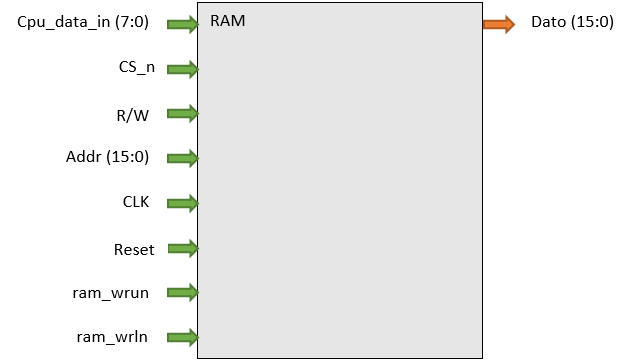```module eth_spram_256x32(

clk, rst, ce, we, oe, addr, di, dato

);

//
// Generic synchronous single-port RAM interface
//
input           clk;  // Clock, rising edge
input           rst;  // Reset, active high
input           cs_n;   // Chip enable input, active high
input           ram_wrun;
input           ram_wrln;
input  [15:0]   cpu_data;   // input data bus
output [15:0]   dato;   // output data bus

//
// Generic single-port synchronous RAM model
//

//
// Generic RAM's registers and wires
//
reg  [15: 0] mem0 [15:0]; // RAM content
reg  [15: 0] mem1; // RAM content
reg  [15:0] mem2; // RAM content
wire cs_n_and_a;
wire cs_n_and_b;
wire wrn_wln;

dato = mem0;
assign cs_n_and_a = ram_wrun and not cs_n;
assign cs_n_and_b = ram_wrln and not cs_n;
assign wrn_wln = ram_wrun or ram_wrln

always@(posedge clk, rst)
if(rst)
mem0 = 0;
mem1 = 0;
mem2 = 0;
begin
if (cs_n_and_a)
mem2[15:8] <=  cpu_data;
else
mem2 <= mem2[mem1][15:8];
if (cs_n_and_b)
mem2[7:0] <=  cpu_data;
else
mem2 <= mem2[mem1][7:0];

if (not cs_n)
else
mem1 <= mem1;

if (wrn_wln)
mem0 <=  mem2[mem1];
end

endmodule
```
```library ieee;
use ieee.std_logic_1164.all;
use ieee.std_logic_arith.all;
use ieee.std_logic_unsigned.all;

library work;
-- use work.memory.all;

entity boot_rom is
port (
addr   : in   std_logic_vector(5 downto 0);
data   : out  std_logic_vector(7 downto 0)
);
end entity boot_rom;

architecture basic of boot_rom is
constant width   : integer := 8;
constant memsize : integer := 64;

type rom_array is array(0 to memsize-1) of std_logic_vector(width-1 downto 0);

constant rom_data : rom_array :=
( "10011100",				             -- \$FFC0 - 9C       RESET RSP
"10100110", "00010001",             -- \$FFC1 - A6 11          LDA #\$11
"10110111", "00010000",             -- \$FFC3 - B7 10          STA ACIACS
"10101110", "00000000",             -- \$FFC5 - AE 00          LDX #\$00
"11010110", "11111111", "11100010", -- \$FFC7 - D6 FFE2  LOOP0 LDA \$FFE0,X
"00100111", "00001000",             -- \$FFCA - 27 08          BEQ INPUT
"00000011", "00010000", "11111101", -- \$FFCC - 03 10 FD LOOP1 BRCLR 1,\$10,LOOP1
"10110111", "00010001",             -- \$FFCF - B7 11          STA ACIADA
"01011100",                         -- \$FFD1 - 5C             INCX
"00100000", "11110011",             -- \$FFD3 - 20 F3          BRA LOOP0
"00000001", "00010000", "11111101", -- \$FFD5 - 01 10 FD INPUT BRCLR 0,\$10,INPUT
"10110110", "00010001",             -- \$FFD7 - B6 11          LDA ACIADA
"11001101", "11111111", "11011111", -- \$FFD9 - CD FFDF        JSR SUBR
"11001100", "11111111", "11000000", -- \$FFDC - CC FFC0        JMP RESET
"10110111", "00010001",             -- \$FFDF - B7 11    SUBR  STA ACIADA
"10000001",                         -- \$FFE1 - 81             RTS
"01001000", "01100101", "01101100", -- \$FFE2 - 48 65 6c MSG   FCC "Hel"
"01101100", "01101111", "00100000", -- \$FFE5 - 6c 6f 20       FCC "lo "
"01010111", "01101111", "01110010", -- \$FFE8 - 57 6f 72       FCC "Wor"
"01101100", "01100100",             -- \$FFEB - 6c 64          FCC "ld"
"00001010", "00001101", "00000000", -- \$FFED - 0a 0d 00       FCB LF,CR,NULL
"11111111", "11000000",             -- \$FFF0 - FF C0          FDB RESET
"11111111", "11000000",             -- \$FFF2 - FF C0          FDB RESET
"11111111", "11000000",             -- \$FFF4 - FF C0          FDB RESET
"11111111", "11000000",             -- \$FFF6 - FF C0          FDB RESET
"11111111", "11000000",             -- \$FFF8 - FF C0          FDB RESET
"11111111", "11000000",             -- \$FFFA - FF C0          FDB RESET
"11111111", "11000000",             -- \$FFFC - FF C0          FDB RESET
"11111111", "11000000"              -- \$FFFE - FF C0          FDB RESET
);
begin
end architecture basic;
```
```library ieee;
use ieee.std_logic_1164.all;
use ieee.std_logic_arith.all;
use ieee.std_logic_unsigned.all;

entity bset_rom is
port (
addr   : in   std_logic_vector(2 downto 0);
data   : out  std_logic_vector(7 downto 0)
);
end entity bset_rom;

architecture basic of bset_rom is
constant width   : integer := 8;
constant memsize : integer := 8;

type bset_rom_array is array(0 to memsize-1) of std_logic_vector(width-1 downto 0);

constant bset_rom_data : bset_rom_array :=
( "00000001",
"00000010",
"00000100",
"00001000",
"00010000",
"00100000",
"01000000",
"10000000"
);
begin
end architecture basic;
```
```library ieee;
use ieee.std_logic_1164.all;
use ieee.std_logic_arith.all;
use ieee.std_logic_unsigned.all;

entity bclr_rom is
port (
addr   : in   std_logic_vector(2 downto 0);
data   : out  std_logic_vector(7 downto 0)
);
end entity bclr_rom;

architecture basic of bclr_rom is
constant width   : integer := 8;
constant memsize : integer := 8;

type bclr_rom_array is array(0 to memsize-1) of std_logic_vector(width-1 downto 0);

constant bclr_rom_data : bclr_rom_array :=
( "11111110",
"11111101",
"11111011",
"11110111",
"11101111",
"11011111",
"10111111",
"01111111"
);
begin
end architecture basic;
```

### 5. Control Unit or Control Bus:

the control unit is a communication link used by the CPU to send data, control signal and address to all the peripherial devices that are contained within the computer. It serves as a medium between the cpu and the rest of the computer. The CPU transmits a variety of control signals to components and devices, One of the main objectives of a bus is to minimize the lines that are needed for communication.

The cpu sends and receives data from the control unit through the ` data_out` and ` cpu_data_in` signal lines respectively. This signals are sent and received from all the peripheral devices, depending on the specific address the cpu wants to communicate with.

The control unit selects this address from signals in Rom, Ram, Timer, Uart, Ioport.

Each peripheral has a specific address on the ` cpu_address bus`. The ` cpu_vma ` when enabled indicates that there is a valid address and the cpu wants to communicate with the peripheral.

Pin Description

PinType Interface Description
Reset Input cpu_reset Reset input (active high)
Cpu_clk Input cpu_clk System Clock
Uart_data_out Input Mini-uart Output data from the UART
Timer_data_out Input timer Output data from the Timer
Ioport_data_out Input ioport Output data from the IOPORT
Rom_data_out Input Rom Output data from the ROM
Ram_data Inout Ram data to/from the RAM
Bus_data Inout Bus data to/from the BUS
CPU_Vma Input CPU Valid Memory Address
CPU_Data_out Input CPU Data output from the CPU
Sysclk Input CPU Clock for timer
Cpu_rw Input CPU System Clock
Cpu_data_in output control unit Cpu data to all peripheral
Baudclock output mini-uart Miniuart RX/TX Clock
Ioport_cs output ioport IOPORT Chip Select
Ram_cs output ram Ram Chip Select
Timer_cs output timer Timer chip select
Uart_cs output mini-uart Mini-uart chip select
Bus_cs output control unit Bus Chip Select
Count_L output Timer Generates Timer clock
Led output Out Indicates circuit is working
Ram_wrl output ram Ram write lower(writes to bit 7:0 of ram address)
Ram_wru output ram Ram write Upper(writes to bit 15:8 of ram address)
Ext_irq output cpu Interrupt
Dcd_n output mini-uart For Debugging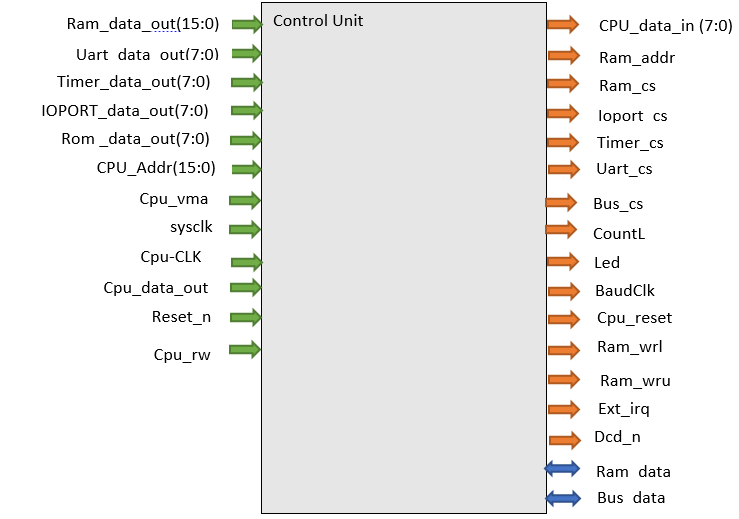```library ieee;
use ieee.std_logic_1164.all;
use IEEE.STD_LOGIC_ARITH.ALL;
use IEEE.STD_LOGIC_UNSIGNED.ALL;
use ieee.numeric_std.all;

entity System05 is
port(
SysClk      : in  Std_Logic;  -- System Clock input
Reset_n     : in  Std_logic;  -- Master Reset input (active low)
LED         : out std_logic;  -- Diagnostic LED Flasher

-- Memory Interface signals
ram_csn     : out Std_Logic;
ram_wrun    : out Std_Logic;
ram_wrln    : out Std_Logic;
ram_addr    : out Std_Logic_Vector(16 downto 0);
ram_data    : inout Std_Logic_Vector(15 downto 0);

-- Stuff on the peripheral board
--  aux_clock   : in  Std_Logic;  -- Extra clock
--	 buzzer      : out Std_Logic;

-- PS/2 Mouse interface
--	 mouse_clock : in  Std_Logic;
--	 mouse_data  : in  Std_Logic;

-- Uart Interface
rxbit       : in  Std_Logic;
txbit       : out Std_Logic;
rts_n       : out Std_Logic;
cts_n       : in  Std_Logic;

-- Keyboard interface
--    kb_clock    : in  Std_Logic;
-- 	kb_data     : in  Std_Logic;

-- CRTC output signals
--	 v_drive     : out Std_Logic;
--    h_drive     : out Std_Logic;
--    blue_lo     : out std_logic;
--    blue_hi     : out std_logic;
--    green_lo    : out std_logic;
--    green_hi    : out std_logic;
--    red_lo      : out std_logic;
--    red_hi      : out std_logic;

-- External Bus
bus_addr     : out   std_logic_vector(15 downto 0);
bus_data     : inout std_logic_vector(7 downto 0);
bus_rw       : out   std_logic;
bus_cs       : out   std_logic;
bus_clk      : out   std_logic;
bus_reset    : out   std_logic;

-- I/O Ports
porta        : inout std_logic_vector(7 downto 0);
portb        : inout std_logic_vector(7 downto 0);
portc        : inout std_logic_vector(7 downto 0);
portd        : inout std_logic_vector(7 downto 0);

-- Timer I/O
--    timer0_in    : in std_logic;
timer0_out   : out std_logic;
--    timer1_in    : in std_logic;
timer1_out   : out std_logic
);
end System05;

-------------------------------------------------------------------------------
-- Architecture for memio Controller Unit
-------------------------------------------------------------------------------
architecture my_computer of System05 is
-----------------------------------------------------------------------------
-- Signals
-----------------------------------------------------------------------------
-- BOOT ROM
signal rom_data_out  : Std_Logic_Vector(7 downto 0);

-- UART Interface signals
signal uart_data_out : Std_Logic_Vector(7 downto 0);
signal uart_cs       : Std_Logic;
signal uart_irq      : Std_Logic;
signal dcd_n         : Std_Logic;

-- I/O Port
signal ioport_data_out : std_logic_vector(7 downto 0);
signal ioport_cs       : std_logic;

-- Timer I/O
signal timer_data_out : std_logic_vector(7 downto 0);
signal timer_cs       : std_logic;
signal timer_irq      : Std_Logic;

-- RAM
signal ram_cs       : std_logic; -- memory chip select
signal ram_wrl      : std_logic; -- memory write lower
signal ram_wru      : std_logic; -- memory write upper
signal ram_data_out : std_logic_vector(7 downto 0);

-- Sequencer Interface signals
signal cpu_reset   : Std_Logic;
signal cpu_clk     : Std_Logic;
signal cpu_rw      : std_logic;
signal cpu_vma     : std_logic;
signal cpu_addr    : Std_Logic_Vector(15 downto 0);
signal cpu_data_in : Std_Logic_Vector(7 downto 0);
signal cpu_data_out: Std_Logic_Vector(7 downto 0);

-- External interrupt input
signal ext_irq     : Std_Logic;

-- Counter signals
signal countL       : std_logic_vector(23 downto 0);
signal BaudCount    : std_logic_vector(4 downto 0);
signal baudclk      : Std_Logic;

-----------------------------------------------------------------
--
-- CPU Core
--
-----------------------------------------------------------------

component cpu05 is
port (
clk       : in  std_logic;
rst       : in  std_logic;
vma       : out std_logic;
rw        : out std_logic;
addr      : out std_logic_vector(15 downto 0);
data_in   : in  std_logic_vector(7 downto 0);
data_out  : out std_logic_vector(7 downto 0);
irq_ext   : in  std_logic;
irq_timer : in  std_logic;
irq_uart  : in  std_logic
);
end component cpu05;

------------------------------------------
--
-- Program memory
--
------------------------------------------

component boot_rom is
port (
addr     : in  Std_Logic_Vector(5 downto 0);  -- 64 byte boot rom
data     : out Std_Logic_Vector(7 downto 0)
);
end component boot_rom;

-----------------------------------------------------------------
--
-- Open Cores Mini UART
--
-----------------------------------------------------------------

component miniUART
port (
clk      : in  Std_Logic;  -- System Clock
rst      : in  Std_Logic;  -- Reset input (active high)
cs       : in  Std_Logic;  -- miniUART Chip Select
rw       : in  Std_Logic;  -- Read / Not Write
irq      : out Std_Logic;  -- Interrupt
Addr     : in  Std_Logic;  -- Register Select
DataIn   : in  Std_Logic_Vector(7 downto 0); -- Data Bus In
DataOut  : out Std_Logic_Vector(7 downto 0); -- Data Bus Out
RxC      : in  Std_Logic;  -- Receive Baud Clock
TxC      : in  Std_Logic;  -- Transmit Baud Clock
RxD      : in  Std_Logic;  -- Receive Data
TxD      : out Std_Logic;  -- Transmit Data
DCD_n    : in  Std_Logic;  -- Data Carrier Detect
CTS_n    : in  Std_Logic;  -- Clear To Send
RTS_n    : out Std_Logic );  -- Request To send
end component;

---------------------------------------
--
-- Three port parallel I/O
--
---------------------------------------

component ioport is
port (
clk      : in std_logic;
rst      : in std_logic;
cs       : in std_logic;
rw       : in std_logic;
addr     : in std_logic_vector(2 downto 0);
data_in  : in std_logic_vector(7 downto 0);
data_out : out std_logic_vector(7 downto 0);
porta_io : inout std_logic_vector(7 downto 0);
portb_io : inout std_logic_vector(7 downto 0);
portc_io : inout std_logic_vector(7 downto 0);
portd_io : inout std_logic_vector(7 downto 0)
);
end component;

----------------------------------------
--
-- Timer module
--
----------------------------------------

component timer is
port (
clk       : in std_logic;
rst       : in std_logic;
cs        : in std_logic;
rw        : in std_logic;
addr      : in std_logic_vector(2 downto 0);
data_in   : in std_logic_vector(7 downto 0);
data_out  : out std_logic_vector(7 downto 0);
irq_out   : out std_logic;
tim0_in   : in std_logic;
tim0_out  : out std_logic;
tim1_in   : in std_logic;
tim1_out  : out std_logic
);
end component;

------------------------------------------
--
-- Global clock buffer for debug
--
------------------------------------------

component BUFG is
port (
i: in std_logic;
o: out std_logic
);
end component;

begin

-----------------------------------------------------------------------------
-- Instantiation of internal components
-----------------------------------------------------------------------------

my_cpu : cpu05 port map (
clk	     => cpu_clk,
rst       => cpu_reset,
vma       => cpu_vma,
rw	     => cpu_rw,
data_in   => cpu_data_in,
data_out  => cpu_data_out,
irq_ext   => ext_irq,
irq_timer => timer_irq,
irq_uart  => uart_irq
);

rom : boot_rom port map (
data       => rom_data_out
);

my_uart  : miniUART port map (
clk	     => cpu_clk,
rst       => cpu_reset,
cs        => uart_cs,
rw        => cpu_rw,
irq       => uart_irq,
Datain    => cpu_data_out,
DataOut   => uart_data_out,
RxC       => baudclk,
TxC       => baudclk,
RxD       => rxbit,
TxD       => txbit,
DCD_n     => dcd_n,
CTS_n     => cts_n,
RTS_n     => rts_n
);

my_ioport  : ioport port map (
clk       => cpu_clk,
rst       => cpu_reset,
cs        => ioport_cs,
rw        => cpu_rw,
data_in   => cpu_data_out,
data_out  => ioport_data_out,
porta_io  => porta,
portb_io  => portb,
portc_io  => portc,
portd_io  => portd
);

my_timer  : timer port map (
clk       => cpu_clk,
rst       => cpu_reset,
cs        => timer_cs,
rw        => cpu_rw,
data_in   => cpu_data_out,
data_out  => timer_data_out,
irq_out   => timer_irq,
tim0_in   => CountL(4),
tim0_out  => timer0_out,
tim1_in   => CountL(6),
tim1_out  => timer1_out
);

bufginst: BUFG port map(
i => countL(0),
o => cpu_clk
);

-- bufginst: BUFG port map(i => SysClk, o => cpu_clk );

----------------------------------------------------------------------
--
--  Processes to read and write memory based on bus signals
--
----------------------------------------------------------------------

memory_decode: process( Reset_n, cpu_clk,
rom_data_out, ram_data_out,
ioport_data_out, timer_data_out, uart_data_out, bus_data )
begin
when "1111111111" =>
cpu_data_in <= rom_data_out;
ram_cs      <= '0';
ioport_cs   <= '0';
timer_cs    <= '0';
uart_cs     <= '0';
bus_cs      <= '0';
when "0000000000" =>
--
-- Decode 64 bytes of I/O space here
--
ram_cs <= '0';
--
-- I/O ports \$0000 - \$0007
--
when "000" =>
cpu_data_in <= ioport_data_out;
ioport_cs   <= cpu_vma;
timer_cs    <= '0';
uart_cs     <= '0';
bus_cs      <= '0';
--
-- Timer \$0008 - \$000F
--
when "001" =>
cpu_data_in <= timer_data_out;
ioport_cs   <= '0';
timer_cs    <= cpu_vma;
uart_cs     <= '0';
bus_cs      <= '0';
--
-- ACIA \$0010 - \$0017
--
when "010" =>
cpu_data_in <= uart_data_out;
ioport_cs   <= '0';
timer_cs    <= '0';
uart_cs     <= cpu_vma;
bus_cs      <= '0';
--
-- Reserved \$0018 - \$003F
--
when others =>
cpu_data_in <= bus_data;
ioport_cs   <= '0';
timer_cs    <= '0';
uart_cs     <= '0';
bus_cs      <= cpu_vma;
end case;
when others =>
cpu_data_in <= ram_data_out;
ram_cs      <= cpu_vma;
ioport_cs   <= '0';
timer_cs    <= '0';
uart_cs     <= '0';
bus_cs      <= '0';
end case;
end process;

--------------------------------------------------------------
--
-- B5 SRAM interface
--
--------------------------------------------------------------
Ram_decode: process( Reset_n, cpu_clk,
ram_cs, ram_wrl, ram_wru, ram_data )
begin
cpu_reset <= not Reset_n;
ram_wrl  <= (not cpu_rw) and cpu_addr(0);
ram_wru  <= (not cpu_rw) and (not cpu_addr(0));
ram_wrln <= not ram_wrl;
ram_wrun <= not ram_wru;
ram_csn  <= not( Reset_n and ram_cs and cpu_clk );

if ram_cs = '1' then

if ram_wrl = '1' then
ram_data(7 downto 0) <= cpu_data_out;
else
ram_data(7 downto 0)  <= "ZZZZZZZZ";
end if;

if ram_wru = '1' then
ram_data(15 downto 8) <= cpu_data_out;
else
ram_data(15 downto 8)  <= "ZZZZZZZZ";
end if;

else
ram_data(7 downto 0)  <= "ZZZZZZZZ";
ram_data(15 downto 8) <= "ZZZZZZZZ";
end if;

ram_data_out(7 downto 0) <= ram_data(15 downto 8);
else
ram_data_out(7 downto 0) <= ram_data(7 downto 0);
end if;

end process;

--
-- CPU bus signals
--
my_bus : process( cpu_clk, cpu_reset, cpu_rw, cpu_addr, cpu_data_out )
begin
bus_clk   <= cpu_clk;
bus_reset <= cpu_reset;
bus_rw    <= cpu_rw;
if( cpu_rw = '1' ) then
bus_data <= "ZZZZZZZZ";
else
bus_data <= cpu_data_out;
end if;
end process;

--
-- flash led to indicate code is working
--
begin
if(SysClk'event and SysClk = '0') then
countL <= countL + 1;
end if;
LED <= countL(21);
end process;

--
-- 57.6 Kbaud * 16 divider for 25 MHz system clock
--
my_baud_clock: process( SysClk )
begin
if(SysClk'event and SysClk = '0') then
if( BaudCount = 26 )	then
BaudCount <= "00000";
else
BaudCount <= BaudCount + 1;
end if;
end if;
baudclk <= BaudCount(4);  -- 25MHz / 27  = 926,000 KHz = 57,870Bd * 16
dcd_n <= '0';
end process;

--
-- tie down inputs and outputs
--
-- CRTC output signals
--
--	 v_drive     <= '0';
--    h_drive     <= '0';
--    blue_lo     <= '0';
--    blue_hi     <= '0';
--    green_lo    <= '0';
--    green_hi    <= '0';
--    red_lo      <= '0';
--    red_hi      <= '0';
--	 buzzer      <= '0';

--
-- tie down unused interrupts
--
ext_irq <= '0';

end my_computer; --===================== End of architecture =======================--
```

### 6. Central Processing Unit (CPU):

The CPU(Central Processing Unit) is made up of the ALU, flags and Control Registers.

ALU:
the `Alu_ctrl` signal is responsible for controlling the operation of the ALU. Each value of the alu_ctrl signal represents a different alu operation. The ALU has a total of 27 instructions, with two registers , the alu_left and the alu_right. And ALU_v as the output.

Instruction Types
The MCU instructions fall into the following four categories:

• Register/Memory instructions
• Bit Manipulation instructions
• Control instructions

1. Register/Memory Instructions
These instructions operate on CPU registers and memory locations. Most of them use two operands. One operand is in either the accumulator or the index register. The CPU finds the other operand in memory.

Alu_ctrl signalMneumonics Instruction
00010 Alu_sub Subtract Memory Byte from Accumulator
00011Alu_sbc Subtract Memory Byte and Carry Bit from Accumulator
00100 Alu_and AND Memory Byte with Accumulator
00101 Alu_ora OR Accumulator with Memory Byte
00110 Alu_eor EXCLUSIVE OR Accumulator with Memory Byte
11000 Alu_ld Load Accumulator/Index Register with Memory Byte
11001 Alu_st Store accumulator/Index Register in Memory
10111 Alu_btst Test for Negative or Zero

These instructions read a memory location or a register, modify its contents, and write the modified value back to the memory location or to the register.

Note :

• Do not use read-modify-write operations on write-only registers.
• Unlike other read-modify-write instructions, BCLR and BSET use only direct addressing.
• TST is an exception to the read-modify-write sequence because it does not write a replacement value.

Alu_ctrl signalMneumonics Instruction
00111 Alu_tst Test for Negative or Zero
01000 Alu_inc Increment
01001 Alu_dec Decrement
01010 Alu_clr Clear Register
01011 Alu_neg Negate (Two’s Complement)
01100 Alu_com Complement (One’s Complement)
01101 Alu_lsr Logical Shift Right
01110 Alu_lsl Logical Shift Left (Same as ASL)
01111 Alu_ror Rotate Right through Carry Bit
10000 Alu_rol Rotate Left through Carry Bit
10001 Alu_asr Arithmetic Shift Right
10101 Alu_bset Bit Set
10110 Alu_bclr Bit Clear

3. Bit Manipulation Instructions
The CPU can set or clear any writable bit in the first 256 bytes of memory, which includes I/O registers and on-chip RAM locations.

Alu_ctrl signalMneumonics Instruction
10101 Alu_bset Bit Set
10110 Alu_bclr Bit Clear

4. Control Instructions
These instructions act on CPU registers and control CPU operation during program execution.

Pin Description

Alu_ctrl signalMneumonics Instruction
10100 Alu_clc Clear carry bit
11010 Alu_nop No Operation
11011 Alu_sec Set carry bit

The cpu has 15 registers:

• Accumulator register(ACR)
• Opcode register(OPR)
• Conditional code register(CCR)
• Index register(IXR)
• Stack pointer register(SPR)
• Program counter register(PCR)
• Memory data register(MDR)
• Interrupt vector register(IVR)
• Data multiplexer register (DMR)
• Alu right register(ARR)
• Alu left register(ALR)

Accumulator (ACR)
The `accumulator` (A) is a general-purpose 8-bit register used to hold operands and results of arithmetic calculations or data manipulations. The accumulator output is either fed as the cpu data out or fed to the alu_register. The accumulator register is control by the ac_ctrl signal. It has four addressing modes: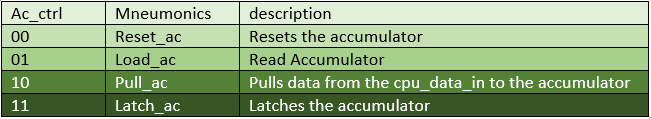Opcode register (OPR):
stores the opcode , the opcode or operation code affects the operation of the cpu the table will be drawn in instruction cycle section. It also contains the address of the bset and bclr data roms. Its output is controlled by the ` op_ctrl` bit. And it has its input fed in directly from the `cpu data_in.` it has four addressing modes: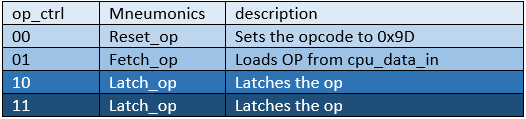Interrupt vector register: (IVR):
This register is controlled by the `iv_ctrl` and it has 6 address modes: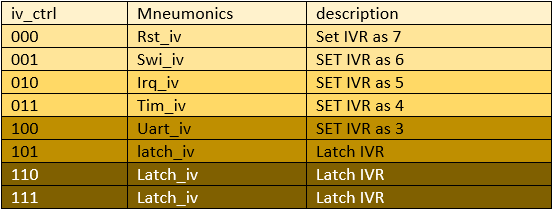Index Register (IXR)
The index register (IXR) is an 8-bit register used by the indexed addressing value to create an effective address. The index register also may be used as a temporary storage area.It can either be fed to the `cpu_data_out `or to the alu register. It is controlled by the ` ix_ctrl` signal, and it has four addressing modes: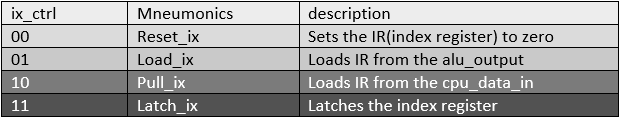Program Counter (PCR):
The program counter (PC) is a 16-bit register that points to the next program address, it is controlled by the `pc_ctrl` and it has seven addressing modes: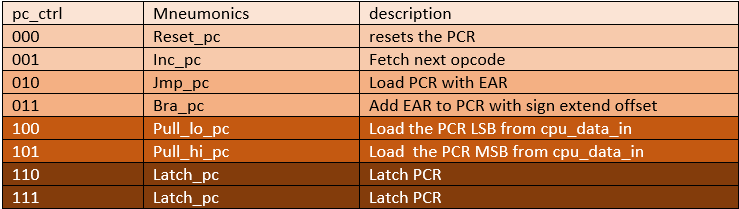Memory data Register (MDR):
JUST like the IXR and ACR the memory data register also stores program data, which can be fed to the alu_register or fed as the `cpu_data_out`. The MDR is controlled by the` md_ctrl` signal and it has four address mode: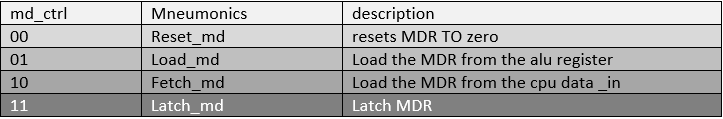This register stores the unique address that the program counter use, it can be used during a jump subroutine or when the program needs to perform a particular cycle outside the program counter. It is controlled by the `ea_ctrl`. It has 7 addressing modes: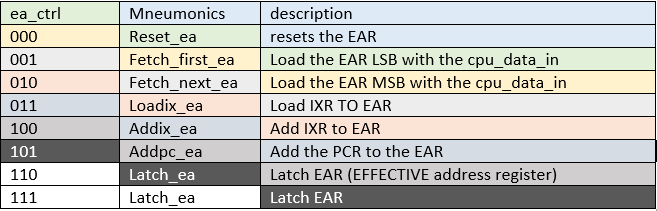Data Output register (DMR)
Also called the Data Output Multiplexer, this register selects what to output as the cpu_output. That is select data to be written to memory, note that that the output is asynchronous. This register is controlled by the ` data_ctrl `bit , and it has five address mode: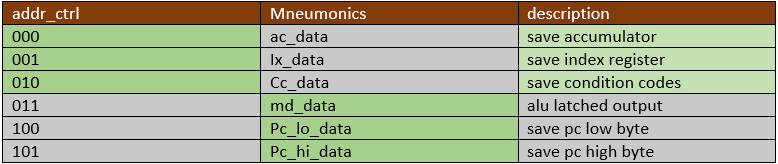Stores the address of the peripheral device the cpu wants to communicate with, it is controlled by the `addr_ctrl` register. It also controls the `RW` and the `VMA` . the vma stands for valid memory access it indicates that the address bus data is valid, this signal is always on except when the address register is reset or when the address register is fetching the interrupt vector hi signal. The `RW` when high indicates that the CPU wants to read , while low it indicates that the cpu wants to write, the cpu is always in the read mode except when it wants to write to the stack or the memory. the `addr_ctrl` register has six addressing modes: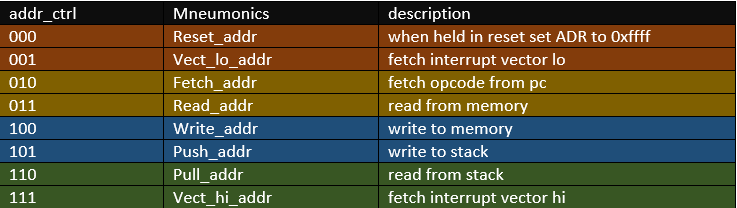Alu register (ALR, ARR):
The alu_registers consists of two 1-byte registers referred to as `alu_left` and `alu_right` they both store Alu data temporary during operation. They are both controlled by the `left_ctrl` and `right_ctrl` respectively. Their address modes are given below: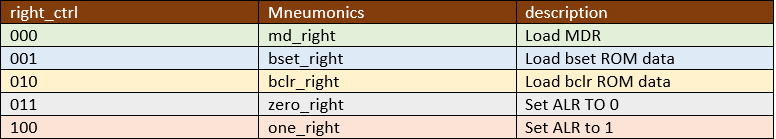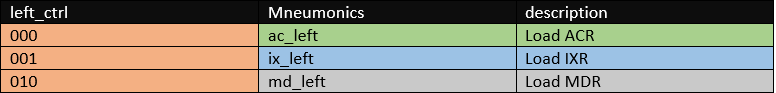Stack Pointer (SPR):
The stack pointer (SP) contains the address of the next available location in an external push-down/pop-up stack. The stack is normally a RAM that may have any location that is convenient .
It contains the address of the next free location on the stack. During an MCU reset or the reset stack pointer (RSP) instruction, the stack pointer is set to location `\$007F`. The stack pointer is then decremented as data is pushed onto the stack and incremented as data is pulled from the stack. The stack register is controlled by the `sp_ctrl` and it has four addressing modes:Condition Code Register (CCR):
The condition code register (CCR) is a 5-bit register in which four bits are used to indicate the results of the instruction executed, and the fifth bit indicates whether interrupts are masked. The CC register is controlled by the `cc_ctrl` signal, and it has four addressing modes: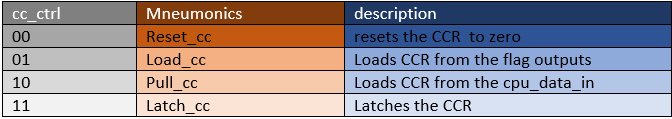The 5-bit register can be tested individually by a program, and specific actions can be taken as a result of their state. Each bit has will be explained below.

The flags:
The cpu has a total of 5flags in the `cc_out` bit also called condition code register. The condition code register (CCR) is a 5-bit register in which four bits are used to indicate the results of the instruction just executed, and the fifth bit indicates whether interrupts are masked. These bits can be tested individually by a program, and specific actions can be taken as a result of their state.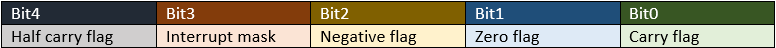Bit4 → Half Carry flag. This bit is set during ADD and ADC operations to indicate that a carry occurred between bits 3 and 4.

Bit3→ Interrupt mask. When this bit is set, the timer and external interrupt are masked (disabled). If an interrupt occurs while this bit is set, the interrupt is latched and processed as soon as the interrupt bit is cleared. N — Negative When set, this bit indicates that the result of the last arithmetic, logical, or data manipulation was negative.

Bit2 → Negative flag. When set, this bit indicates that the result of the last arithmetic, logical, or data manipulation was a negative value.

Bit1 → Zero flag. When set, this bit indicates that the result of the last arithmetic, logical, or data manipulation was 0.

Bit0 → Carry/Borrow flag. When set, this bit indicates that a carry or borrow out of the arithmetic logical unit (ALU) occurred during the last arithmetic operation. This bit also is affected during bit test and branch instructions and during shifts and rotates.

The (CPU) Central Processing Unit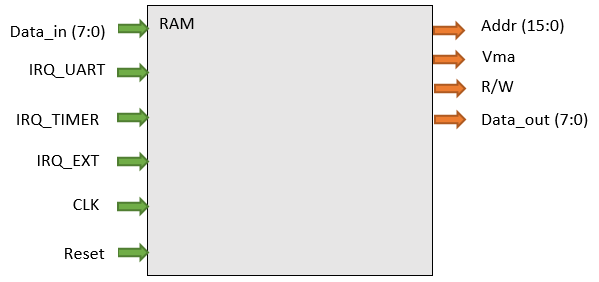Pin Description

PinType Interface Description
Data_in Input Control_unit CPU input data
Irq_uart Input mini-uart Interrupt request from UART
Irq_timer Input Timer Interrupt request from Timer
Irq_ext Input Control_unit Interrupt request from Control unit
Reset Input Reset Reset input (active high)
Clock Input Input cpu_clk System Clock
Vma Output Control unit Valid Memory Address
Data_out Output Peripherals Output Data from CPU

### Instruction Cycle

The Instruction cycle (also known as the fetch–decode–execute cycle, or simply the fetch-execute cycle) is the cycle that the central processing unit (CPU) follows from boot-up until the computer has shut down in order to process instructions. A program residing in the memory unit of a computer consists of a sequence of instructions.

These instructions are executed by the processor by going through a cycle for each instruction. Instructions are processed under direction of the cpu in step-by-step manner. Each step is referred to as a phase. The cycle begins as soon as power is applied to the system, with an initial OPR value that is predefined by the system's architecture. Typically, this address points to a set of instructions in read-only memory (ROM), which begins the process of loading (or booting) the operating system.

There are 42 fundamental phases of the instruction cycle: The chart if given below:

```library ieee;
use ieee.std_logic_1164.all;
use ieee.std_logic_unsigned.all;

entity cpu05 is
port (
clk       : in  std_logic;
rst       : in  std_logic;
vma       : out std_logic;
rw        : out std_logic;
addr      : out std_logic_vector(15 downto 0);
data_in   : in  std_logic_vector(7 downto 0);
data_out  : out std_logic_vector(7 downto 0);
irq_ext   : in  std_logic;
irq_timer : in  std_logic;
irq_uart  : in  std_logic );
end;

architecture cpu_arch of cpu05 is
type state_type is (reset_state, reset1_state, reset2_state,
fetch_state, decode_state, exec_state, halt_state,
brbit_state,
branch_state, bsr_state, jsr_state, jsr1_state, jmp_state,
swi_state, stop_state,
rti_state, rti_cc_state, rti_ac_state, rti_ix_state, rti_pch_state, rti_pcl_state,
rts_state, rts_pch_state, rts_pcl_state,
wait_state, wait1_state, wait2_state, wait3_state, wait4_state,
int_state, int1_state, int2_state, int3_state, int4_state, int5_state, int6_state,
dir_state, ext_state, ix2_state, ix1_state, ix0_state,
write_state );
type data_type is (ac_data, ix_data, cc_data, md_data, pc_lo_data, pc_hi_data );
type pc_type is (reset_pc, latch_pc, inc_pc, jmp_pc, bra_pc, pull_lo_pc, pull_hi_pc );
type op_type is (reset_op, latch_op, fetch_op );
type ac_type is (reset_ac, latch_ac, load_ac, pull_ac );
type ix_type is (reset_ix, latch_ix, load_ix, pull_ix );
type sp_type is (reset_sp, latch_sp, load_sp, inc_sp, dec_sp );
type cc_type is (reset_cc, latch_cc, load_cc, pull_cc );
type md_type is (reset_md, latch_md, load_md, fetch_md );
type iv_type is (latch_iv, rst_iv, swi_iv, irq_iv, tim_iv, uart_iv );
type left_type  is (ac_left, ix_left, md_left );
type right_type is (md_right, bset_right, bclr_right, zero_right, one_right );
alu_and, alu_ora, alu_eor,
alu_tst, alu_inc, alu_dec, alu_clr, alu_neg, alu_com,
alu_lsr, alu_lsl, alu_ror, alu_rol, alu_asr,
alu_sei, alu_cli, alu_sec, alu_clc,
alu_bset, alu_bclr, alu_btst,
alu_ld, alu_st, alu_nop );

constant HFLAG : integer := 4;
constant IFLAG : integer := 3;
constant NFLAG : integer := 2;
constant ZFLAG : integer := 1;
constant CFLAG : integer := 0;

--
-- internal registers
--
signal ac:          std_logic_vector(7 downto 0);	-- accumulator
signal ix:          std_logic_vector(7 downto 0);  -- index register
signal sp:          std_logic_vector(6 downto 0);  -- stack pointer
signal cc:          std_logic_vector(4 downto 0);  -- condition codes from alu
signal pc:	        std_logic_vector(15 downto 0); -- program counter for opcode access
signal ea:          std_logic_vector(15 downto 0); -- effective addres for memory access
signal op:          std_logic_vector(7 downto 0);  -- opcode register
signal md:          std_logic_vector(7 downto 0);  -- memory data
signal iv:          std_logic_vector(2 downto 0);  -- interrupt vector number
signal state:       state_type;

--
-- unregistered signals
-- (combinational logic)
--
signal alu_left:    std_logic_vector(8 downto 0);  -- alu left input (bit 8 for carry)
signal alu_right:   std_logic_vector(8 downto 0);  -- alu right input
signal alu_out:     std_logic_vector(8 downto 0);  -- alu result output (unlatched)
signal cc_out:      std_logic_vector(4 downto 0);  -- alu condition code outout (unlatched)
signal bset_data_out: std_logic_vector(7 downto 0);
signal bclr_data_out: std_logic_vector(7 downto 0);
signal next_state:  state_type;

--
-- Syncronous Register Controls
--
signal ac_ctrl:     ac_type;
signal ix_ctrl:     ix_type;
signal sp_ctrl:     sp_type;
signal cc_ctrl:     cc_type;
signal pc_ctrl:     pc_type;
signal ea_ctrl:     ea_type;
signal op_ctrl:     op_type;
signal md_ctrl:     md_type;
signal iv_ctrl:     iv_type;

--
-- Asynchronous Multiplexer Controls
--
signal data_ctrl: data_type;   -- data output mutiplexer
signal left_ctrl:   left_type;       -- Left ALU input
signal right_ctrl:  right_type;     -- Right ALU input
signal alu_ctrl:    alu_type;        -- ALU opeartion

--
-- bit set decoder table
--
component bset_rom is
port (
addr     : in  Std_Logic_Vector(2 downto 0);
data     : out Std_Logic_Vector(7 downto 0)
);
end component bset_rom;
--
-- bit clear decoder table
--
component bclr_rom is
port (
addr     : in  Std_Logic_Vector(2 downto 0);
data     : out Std_Logic_Vector(7 downto 0)
);
end component bclr_rom;

begin

rom_set : bset_rom port map (
data       => bset_data_out
);

rom_clear : bclr_rom port map (
data       => bclr_data_out
);

----------------------------------
--
-- opcode register
--
----------------------------------

op_reg: process( clk, op_ctrl, data_in, op )
begin
if clk'event and clk = '0' then
case op_ctrl is
when reset_op =>
op <= "10011101";	-- reset with NOP
when fetch_op =>
op <= data_in;
when others =>
--	 when latch_op =>
op <= op;
end case;
end if;
end process;

-----------------------------------
--
-- accumulator
--
------------------------------------
ac_reg : process( clk, ac_ctrl, alu_out, ac, ix, data_in )
begin
if clk'event and clk = '0' then
case ac_ctrl is
when reset_ac =>             -- released from reset
ac <= "00000000";
when load_ac =>               -- single or dual operation
ac <= alu_out(7 downto 0);
when pull_ac =>                -- read acc / increment sp
ac <= data_in;
when others =>               -- halt on undefine states
--	  when latch_ac =>             -- no operation on acc
ac <= ac;
end case;
end if;
end process;

------------------------------------
--
-- condition code register
--
------------------------------------
cc_reg : process( clk, cc_ctrl, cc_out  )
begin
if clk'event and clk = '0' then
case cc_ctrl is
when reset_cc =>             -- released from reset
cc <= "00000";
when load_cc =>               -- single or dual operation
cc <= cc_out;
when pull_cc =>                -- read cc / increment sp
cc <= data_in(4 downto 0);
when others =>               -- halt on undefine states
--	  when latch_cc =>             -- no operation on acc
cc <= cc;
end case;
end if;
end process;

------------------------------------
--
-- index register
--
------------------------------------
ix_reg : process( clk, ix_ctrl, alu_out, ac, ix, data_in )
begin
if clk'event and clk = '0' then
case ix_ctrl is
when reset_ix =>                 -- released from reset
ix <= "00000000";
when load_ix =>                   -- execute /  = alu out
ix <= alu_out(7 downto 0);
when pull_ix =>                    -- read ixreg / increment sp
ix <= data_in;
when others =>
--	  when latch_ix =>                 -- no change in ix
ix <= ix;
end case;
end if;
end process;

------------------------------------
--
-- stack pointer
--
------------------------------------
sp_reg : process( clk, sp_ctrl, sp )
begin
if clk'event and clk = '0' then
case sp_ctrl is
when reset_sp =>                 -- released from reset
sp <= "1111111";
when inc_sp =>                   -- pop registers
sp <= sp + 1;
when dec_sp =>                   -- push registes
sp <= sp - 1;
when others =>
--	  when latch_sp =>                 -- no change in sp
sp <= sp;
end case;
end if;
end process;

------------------------------------
--
-- program counter
--
------------------------------------
pc_reg : process( clk, pc_ctrl, pc, ea, data_in )
variable offset : std_logic_vector(15 downto 0);
begin
if clk'event and clk = '0' then
case pc_ctrl is
when reset_pc =>                 -- released from reset
pc <= "0000000000000000";
when inc_pc =>                   -- fetch next opcode
pc <= pc + 1;
pc <= ea;
if ea(7) = '0' then				  -- sign extend offset
offset := "00000000" & ea(7 downto 0);
else
offset := "11111111" & ea(7 downto 0);
end if;
pc <= pc + offset;
when pull_lo_pc =>               -- load pc lo byte from memory
pc(15 downto 8) <= pc(15 downto 8);
pc(7 downto 0)  <= data_in;
when pull_hi_pc =>               -- load pc hi byte from memory
pc(15 downto 8) <= data_in;
pc(7 downto 0)  <= pc(7 downto 0);
when others =>                   -- halt on undefine states
--	  when latch_pc =>                 -- no change in pc
pc <= pc;
end case;
end if;
end process;

------------------------------------
--
--
------------------------------------
ea_reg: process( clk, ea_ctrl, ea, pc, ix, data_in )
variable offset : std_logic_vector(15 downto 0);
begin
if clk'event and clk = '0' then
case ea_ctrl is
when reset_ea =>                     -- released from reset / fetch
ea <= "0000000000000000";
ea <= "00000000" & ix;
if ea(7) = '0' then				  -- sign extend offset
offset := "00000000" & ea(7 downto 0);
else
offset := "11111111" & ea(7 downto 0);
end if;
ea <= offset + pc;
ea <= ea + ("00000000" & ix );
when fetch_first_ea =>               -- load ea lo byte from memory
ea(15 downto 8) <= "00000000";
ea(7 downto 0) <= data_in;
when fetch_next_ea =>               -- load ea with second from memory
ea(15 downto 8) <= ea(7 downto 0);
ea(7 downto 0) <= data_in;
when others =>                   -- halt on undefine states
--	  when latch_ea =>                 -- no change in ea
ea <= ea;
end case;
end if;
end process;

----------------------------------
--
-- memory data register
-- latch memory byte input
--
----------------------------------

md_reg: process( clk, md_ctrl, data_in, md )
begin
if clk'event and clk = '0' then
case md_ctrl is
when reset_md =>
md <= "00000000";
when latch_md =>
md <= md;
when load_md =>                  -- latch alu output
md <= alu_out(7 downto 0);
when fetch_md =>
md <= data_in;
when others =>
null;
end case;
end if;
end process;

----------------------------------
--
-- interrupt vector register
--
----------------------------------

iv_reg: process( clk, iv_ctrl, iv )
begin
if clk'event and clk = '0' then
case iv_ctrl is
when rst_iv =>
iv <= "111"; -- \$FFFE/\$FFFF
when swi_iv =>
iv <= "110"; -- \$FFFC/\$FFFD
when irq_iv =>
iv <= "101"; -- \$FFFA/\$FFFB
when tim_iv =>
iv <= "100"; -- \$FFF8/\$FFF9
when uart_iv =>
iv <= "011"; -- \$FFFA/\$FFFB
when others =>
--	 when latch_iv =>
iv <= iv;
end case;
end if;
end process;

----------------------------------
--
-- Work out which register to apply to the address bus
-- Note that the multiplexer output is asyncronous
--
----------------------------------

begin
when reset_addr =>                 -- when held in reset
vma  <= '0';
rw   <= '1';
when fetch_addr =>                 -- fetch opcode from pc
vma  <= '1';
rw   <= '1';
vma  <= '1';
rw   <= '1';
when write_addr =>                 -- write to memory
vma  <= '1';
rw   <= '0';
when push_addr =>                  -- write to stack
vma  <= '1';
rw   <= '0';
vma  <= '1';
rw   <= '1';

when vect_hi_addr =>               -- fetch interrupt vector hi
addr <= "111111111111" & iv & "0";
vma  <= '1';
rw   <= '1';
when vect_lo_addr =>               -- fetch interrupt vector lo
addr <= "111111111111" & iv & "1";
vma  <= '1';
rw   <= '1';
when others =>                   -- undefined all high
vma  <= '0';
rw   <= '1';
end case;
end process;

----------------------------------
--
-- Data Output Multiplexer
-- select data to be written to memory
-- note that the output is asynchronous
--
----------------------------------

mux_data: process( clk, data_ctrl, md, pc, cc, ac, ix )
variable data_out_v : std_logic_vector(7 downto 0);
begin
case data_ctrl is
when ac_data =>                   -- save accumulator
data_out <= ac;
when ix_data =>                   -- save index register
data_out <= ix;
when cc_data =>                   -- save condition codes
data_out <= "000" & cc;
when others =>
--  	 when md_data =>                  -- alu latched output
data_out <= md;
when pc_lo_data =>                  -- save pc low byte
data_out <= pc(7 downto 0);
when pc_hi_data =>
data_out <= pc(15 downto 8); -- save pc high byte

end case;
end process;

----------------------------------
--
-- alu left mux
-- asynchronous input as register is already latched
--
----------------------------------

mux_left: process( clk, left_ctrl, ac, ix, md )
begin
case left_ctrl is
when ac_left =>
alu_left <= "0" & ac; -- dual op argument
when ix_left =>
alu_left <= "0" & ix; -- dual op argument
when md_left =>
alu_left <= "0" & md;
when others =>
alu_left <= "000000000";
end case;
end process;

----------------------------------
--
-- alu right mux
-- asynchronous input as register is already latched
--
----------------------------------

mux_right: process( clk, right_ctrl, data_in, bset_data_out, bclr_data_out, md )
begin
case right_ctrl is
when others =>
--	 when md_right =>
alu_right <= "0" & md; -- dual op argument
when bset_right =>
alu_right <= "0" & bset_data_out;
when bclr_right =>
alu_right <= "0" & bclr_data_out;
when zero_right =>
alu_right <= "000000000";
when one_right =>
alu_right <= "000000001";

end case;
end process;

----------------------------------
--
-- Arithmetic Logic Unit
--
----------------------------------

mux_alu: process( clk, alu_ctrl, cc, alu_left, alu_right )
variable alu_v   : std_logic_vector(8 downto 0);
variable low_v   : std_logic_vector(4 downto 0);
variable high_v  : std_logic_vector(4 downto 0);
begin

case alu_ctrl is
when alu_bset =>
alu_v := alu_left or alu_right; 	-- bit
when alu_bclr =>
alu_v := alu_left and alu_right; 	-- bclr
when alu_btst =>
alu_v := alu_left and alu_right; 	-- tst
low_v   := ("0" & alu_left(3 downto 0)) + ("0" & alu_right(3 downto 0));
high_v  := ("0" & alu_left(7 downto 4)) + ("0" & alu_right(7 downto 4)) + low_v(4);
alu_v   := high_v(4 downto 0) & low_v(3 downto 0); 	-- add
low_v   := ("0" & alu_left(3 downto 0)) + ("0" & alu_right(3 downto 0)) + ("0000" & cc(CFLAG));
high_v  := ("0" & alu_left(7 downto 4)) + ("0" & alu_right(7 downto 4)) + low_v(4);
alu_v   := high_v(4 downto 0) & low_v(3 downto 0); 	-- adc
when alu_sub =>
alu_v   := alu_left - alu_right; 	-- sub / cmp
when alu_sbc =>
alu_v   := alu_left - alu_right - ("00000000" & cc(CFLAG)); 	-- sbc
when alu_and =>
alu_v   := alu_left and alu_right; 	-- and/bit
when alu_ora =>
alu_v   := alu_left or alu_right; 	-- or
when alu_eor =>
alu_v   := alu_left xor alu_right; 	-- eor/xor
when alu_lsl =>
alu_v   := alu_left(7 downto 0) & "0"; 	-- lsl
when alu_lsr =>
alu_v   := alu_left(0) & "0" & alu_left(7 downto 1); 	-- lsr
when alu_asr =>
alu_v   := alu_left(0) & alu_left(7) & alu_left(7 downto 1); 	-- asr
when alu_rol =>
alu_v   := alu_left(7 downto 0) & cc(CFLAG); 	-- rol
when alu_ror =>
alu_v   := alu_left(0) & cc(CFLAG) & alu_left(7 downto 1); 	-- ror
when alu_inc =>
alu_v   := alu_left + "000000001"; 	-- inc
when alu_dec =>
alu_v   := alu_left(8 downto 0) - "000000001"; -- dec
when alu_neg =>
alu_v   := "000000000" - alu_left(8 downto 0); 	-- neg
when alu_com =>
alu_v   := not alu_left(8 downto 0); 	-- com
when alu_clr =>
alu_v  := "000000000"; 	   -- clr
when alu_ld =>
alu_v  := alu_right(8 downto 0);
when alu_st =>
alu_v  := alu_left(8 downto 0);
when others =>
alu_v  := alu_left(8 downto 0); -- nop
end case;

--
-- carry bit
--
case alu_ctrl is
alu_lsl | alu_lsr | alu_rol | alu_ror | alu_asr |
alu_neg | alu_com =>
cc_out(CFLAG) <= alu_v(8);
when alu_btst =>
cc_out(CFLAG) <= not( alu_v(7) or alu_v(6) or alu_v(5) or alu_v(4) or
alu_v(3) or alu_v(2) or alu_v(1) or alu_v(0) );
when alu_sec =>
cc_out(CFLAG) <= '1';
when alu_clc =>
cc_out(CFLAG) <= '0';
when others =>
cc_out(CFLAG) <= cc(CFLAG);
end case;

--
-- Zero flag
--
case alu_ctrl is
alu_and | alu_ora | alu_eor |
alu_lsl | alu_lsr | alu_rol | alu_ror | alu_asr |
alu_inc | alu_dec | alu_neg | alu_com | alu_clr |
alu_ld  | alu_st =>
cc_out(ZFLAG) <= not( alu_v(7) or alu_v(6) or alu_v(5) or alu_v(4) or
alu_v(3) or alu_v(2) or alu_v(1) or alu_v(0) );
when others =>
cc_out(ZFLAG) <= cc(ZFLAG);
end case;

--
-- negative flag
--
case alu_ctrl is
alu_and | alu_ora | alu_eor |
alu_lsl | alu_lsr | alu_rol | alu_ror | alu_asr |
alu_inc | alu_dec | alu_neg | alu_com | alu_clr |
alu_ld  | alu_st =>
cc_out(NFLAG) <= alu_v(7);
when others =>
cc_out(NFLAG) <= cc(NFLAG);
end case;

--
--
case alu_ctrl is
when alu_sei =>
cc_out(IFLAG) <= '1';               -- set interrupt mask
when alu_cli =>
cc_out(IFLAG) <= '0';               -- clear interrupt mask
when others =>
cc_out(IFLAG) <= cc(IFLAG);             -- interrupt mask
end case;

--
-- Half Carry flag
--
case alu_ctrl is
cc_out(HFLAG) <= low_v(4);
when others =>
cc_out(HFLAG) <= cc(HFLAG);
end case;

alu_out <= alu_v;

end process;

------------------------------------
--
-- state sequencer
--
------------------------------------

sequencer : process( state, data_in,
ac, cc, ix, sp, ea, md, pc, op,
irq_ext, irq_timer, irq_uart )
begin
case state is
--
-- RESET:
-- Here if processor held in reset
-- On release of reset go to RESET1
--
when reset_state =>        -- release from reset
ac_ctrl    <= reset_ac;
cc_ctrl    <= reset_cc;
ix_ctrl    <= reset_ix;
sp_ctrl    <= reset_sp;
pc_ctrl    <= reset_pc;
op_ctrl    <= reset_op;
ea_ctrl    <= reset_ea;
md_ctrl    <= reset_md;
iv_ctrl    <= rst_iv;
left_ctrl  <= ac_left;        -- Left ALU input
right_ctrl <= md_right;       -- Right ALU input
alu_ctrl   <= alu_nop;        -- ALU opeartion
data_ctrl  <= md_data;        -- data output mutiplexer
next_state <= reset1_state;
--
-- RESET1:
-- address bus = reset vector hi
-- Load PC high with high byte
-- go to RESET2
--
when reset1_state =>        -- fetch hi reset vector
ac_ctrl    <= latch_ac;
cc_ctrl    <= latch_cc;
ix_ctrl    <= latch_ix;
sp_ctrl    <= latch_sp;
pc_ctrl    <= pull_hi_pc;
op_ctrl    <= latch_op;
ea_ctrl    <= latch_ea;
md_ctrl    <= latch_md;
iv_ctrl    <= latch_iv;
left_ctrl  <= ac_left;       -- Left ALU input
right_ctrl <= md_right;      -- Right ALU input
alu_ctrl   <= alu_nop;       -- ALU opeartion
data_ctrl  <= md_data;       -- data output mutiplexer
next_state <= reset2_state;
--
-- RESET2:
-- address bus = reset vector lo
-- Load PC low with low byte
-- go to FETCH
--
when reset2_state =>        -- fetch low reset vector
ac_ctrl    <= latch_ac;
cc_ctrl    <= latch_cc;
ix_ctrl    <= latch_ix;
sp_ctrl    <= latch_sp;
pc_ctrl    <= pull_lo_pc;
op_ctrl    <= latch_op;
ea_ctrl    <= latch_ea;
md_ctrl    <= latch_md;
iv_ctrl    <= latch_iv;
left_ctrl  <= ac_left;       -- Left ALU input
right_ctrl <= md_right;      -- Right ALU input
alu_ctrl   <= alu_nop;       -- ALU opeartion
data_ctrl  <= md_data;       -- data output mutiplexer
next_state <= fetch_state;
--
-- FETCH:
-- fetch opcode,
-- clear the effective address (ea) register
-- goto DECODE
--
when fetch_state =>         -- fetch instruction
case op(7 downto 4) is
--				 when "0000" =>				-- BRSET/ BRCLR
--				   null;
--				 when "0001" =>				-- BSET/ BCLR
--				   null;
--				 when "0010" =>				-- BR conditional
--				   null;
--				 when "0011" =>				--	single op direct
--				   null;
when "0100" =>            -- single op accum
ix_ctrl    <= latch_ix;
sp_ctrl    <= latch_sp;
left_ctrl  <= ac_left;
case op( 3 downto 0 ) is
when "0000" =>            -- neg
right_ctrl <= zero_right;
alu_ctrl   <= alu_neg;
when "0011" =>            -- com
right_ctrl <= zero_right;
alu_ctrl   <= alu_com;
when "0100" =>            -- lsr
right_ctrl <= zero_right;
alu_ctrl   <= alu_lsr;
when "0110" =>            -- ror
right_ctrl <= zero_right;
alu_ctrl   <= alu_ror;
when "0111" =>            -- asr
right_ctrl <= zero_right;
alu_ctrl   <= alu_asr;
when "1000" =>            -- lsl
right_ctrl <= zero_right;
alu_ctrl   <= alu_lsl;
when "1001" =>            -- rol
right_ctrl <= zero_right;
alu_ctrl   <= alu_rol;
when "1010" =>            -- dec
right_ctrl <= one_right;
alu_ctrl   <= alu_dec;
when "1011" =>            -- undef
right_ctrl <= zero_right;
alu_ctrl   <= alu_nop;
when "1100" =>            -- inc
right_ctrl <= one_right;
alu_ctrl   <= alu_inc;
when "1101" =>            -- tst
right_ctrl <= zero_right;
alu_ctrl   <= alu_tst;
when "1110" =>            -- undef
right_ctrl <= zero_right;
alu_ctrl   <= alu_nop;
when "1111" =>            -- clr
right_ctrl <= zero_right;
alu_ctrl   <= alu_clr;
when others =>
right_ctrl <= zero_right;
alu_ctrl   <= alu_nop;
end case;

when "0101" =>            -- single op ix reg
ac_ctrl    <= latch_ac;
sp_ctrl    <= latch_sp;
left_ctrl  <= ix_left;
case op( 3 downto 0 ) is
when "0000" =>            -- neg
right_ctrl <= zero_right;
alu_ctrl   <= alu_neg;
when "0011" =>            -- com
right_ctrl <= zero_right;
alu_ctrl   <= alu_com;
when "0100" =>            -- lsr
right_ctrl <= zero_right;
alu_ctrl   <= alu_lsr;
when "0110" =>            -- ror
right_ctrl <= zero_right;
alu_ctrl   <= alu_ror;
when "0111" =>            -- asr
right_ctrl <= zero_right;
alu_ctrl   <= alu_asr;
when "1000" =>            -- lsl
right_ctrl <= zero_right;
alu_ctrl   <= alu_lsl;
when "1001" =>            -- rol
right_ctrl <= zero_right;
alu_ctrl   <= alu_rol;
when "1010" =>            -- dec
right_ctrl <= one_right;
alu_ctrl   <= alu_dec;
when "1011" =>            -- undef
right_ctrl <= zero_right;
alu_ctrl   <= alu_nop;
when "1100" =>            -- inc
right_ctrl <= one_right;
alu_ctrl   <= alu_inc;
when "1101" =>            -- tst
right_ctrl <= zero_right;
alu_ctrl   <= alu_tst;
when "1110" =>            -- undef
right_ctrl <= zero_right;
alu_ctrl   <= alu_nop;
when "1111" =>            -- clr
right_ctrl <= zero_right;
alu_ctrl   <= alu_clr;
when others =>
right_ctrl <= zero_right;
alu_ctrl   <= alu_nop;
end case;
--           when "0110" =>            -- single op IX1
--             null;
--           when "0111" =>            -- single op IX0
--             null;
--           when "1000" =>            -- inherent stack operators
--             null;
when "1001" =>            -- inherent operators
case op( 3 downto 0 ) is
--				   when "0000" =>            -- undef
--					  null;
--     		   when "0001" =>            -- undef
--					  null;
-- 				when "0010" =>            -- undef
--					  null;
--				   when "0011" =>            -- undef
--					  null;
--				   when "0100" =>            -- undef
--					  null;
--				   when "0101" =>            -- undef
--					  null;
--				   when "0110" =>            -- undef
--					  null;
when "0111" =>            -- tax
ac_ctrl    <= latch_ac;
cc_ctrl    <= latch_cc;
sp_ctrl    <= latch_sp;
left_ctrl  <= ac_left;
right_ctrl <= bclr_right;
alu_ctrl   <= alu_st;

when "1000" =>            -- clc
ac_ctrl    <= latch_ac;
ix_ctrl    <= latch_ix;
sp_ctrl    <= latch_sp;
left_ctrl  <= ac_left;
right_ctrl <= bclr_right;
alu_ctrl   <= alu_clc;

when "1001" =>            -- sec
ac_ctrl    <= latch_ac;
ix_ctrl    <= latch_ix;
sp_ctrl    <= latch_sp;
left_ctrl  <= ac_left;
right_ctrl <= bclr_right;
alu_ctrl   <= alu_sec;

when "1010" =>            -- cli
ac_ctrl    <= latch_ac;
ix_ctrl    <= latch_ix;
sp_ctrl    <= latch_sp;
left_ctrl  <= ac_left;
right_ctrl <= bclr_right;
alu_ctrl   <= alu_cli;

when "1011" =>            -- sei
ac_ctrl    <= latch_ac;
ix_ctrl    <= latch_ix;
sp_ctrl    <= latch_sp;
left_ctrl  <= ac_left;
right_ctrl <= bclr_right;
alu_ctrl   <= alu_sei;

when "1100" =>            -- rsp
ac_ctrl    <= latch_ac;
cc_ctrl    <= latch_cc;
ix_ctrl    <= latch_ix;
sp_ctrl    <= reset_sp;
left_ctrl  <= ac_left;
right_ctrl <= bclr_right;
alu_ctrl   <= alu_nop;

--				   when "1101" =>            -- nop
--					  null;
--				   when "1110" =>            -- undef
--					  null;
when "1111" =>            -- txa
cc_ctrl    <= latch_cc;
ix_ctrl    <= latch_ix;
sp_ctrl    <= latch_sp;
left_ctrl  <= ix_left;
right_ctrl <= bclr_right;
alu_ctrl   <= alu_st;

when others =>
ac_ctrl    <= latch_ac;
cc_ctrl    <= latch_cc;
ix_ctrl    <= latch_ix;
sp_ctrl    <= latch_sp;
left_ctrl  <= ix_left;
right_ctrl <= bclr_right;
alu_ctrl   <= alu_nop;
end case;
--
--
when "1010" |            -- dual op imm
"1011" |            -- dual op dir
"1100" |            -- dual op ext
"1101" |            -- dual op ix2
"1110" |            -- dual op ix1
"1111" =>           -- dual op ix0
case op( 3 downto 0 ) is
when "0000" =>            -- sub
ix_ctrl    <= latch_ix;
sp_ctrl    <= latch_sp;
left_ctrl  <= ac_left;
right_ctrl <= md_right;
alu_ctrl   <= alu_sub;
when "0001" =>            -- cmp
ac_ctrl    <= latch_ac;
ix_ctrl    <= latch_ix;
sp_ctrl    <= latch_sp;
left_ctrl  <= ac_left;
right_ctrl <= md_right;
alu_ctrl   <= alu_sub;
when "0010" =>            -- sbc
ix_ctrl    <= latch_ix;
sp_ctrl    <= latch_sp;
left_ctrl  <= ac_left;
right_ctrl <= md_right;
alu_ctrl   <= alu_sbc;
when "0011" =>            -- cpx
ac_ctrl    <= latch_ac;
ix_ctrl    <= latch_ix;
sp_ctrl    <= latch_sp;
left_ctrl  <= ix_left;
right_ctrl <= md_right;
alu_ctrl   <= alu_sub;
when "0100" =>            -- and
ix_ctrl    <= latch_ix;
sp_ctrl    <= latch_sp;
left_ctrl  <= ac_left;
right_ctrl <= md_right;
alu_ctrl   <= alu_and;
when "0101" =>            -- bit
ac_ctrl    <= latch_ac;
ix_ctrl    <= latch_ix;
sp_ctrl    <= latch_sp;
left_ctrl  <= ac_left;
right_ctrl <= md_right;
alu_ctrl   <= alu_and;
when "0110" =>            -- lda
ix_ctrl    <= latch_ix;
sp_ctrl    <= latch_sp;
left_ctrl  <= ac_left;
right_ctrl <= md_right;
alu_ctrl   <= alu_ld;
when "0111" =>            -- sta
ac_ctrl    <= latch_ac;
ix_ctrl    <= latch_ix;
sp_ctrl    <= latch_sp;
left_ctrl  <= ac_left;
right_ctrl <= md_right;
alu_ctrl   <= alu_st;
when "1000" =>            -- eor
ix_ctrl    <= latch_ix;
sp_ctrl    <= latch_sp;
left_ctrl  <= ac_left;
right_ctrl <= md_right;
alu_ctrl   <= alu_eor;
ix_ctrl    <= latch_ix;
sp_ctrl    <= latch_sp;
left_ctrl  <= ac_left;
right_ctrl <= md_right;
when "1010" =>            -- ora
ix_ctrl    <= latch_ix;
sp_ctrl    <= latch_sp;
left_ctrl  <= ac_left;
right_ctrl <= md_right;
alu_ctrl   <= alu_ora;
ix_ctrl    <= latch_ix;
sp_ctrl    <= latch_sp;
left_ctrl  <= ac_left;
right_ctrl <= md_right;
--				   when "1100" =>            -- jmp
--					  null;
--				   when "1101" =>            -- jsr
--					  null;
when "1110" =>            -- ldx
ac_ctrl    <= latch_ac;
sp_ctrl    <= latch_sp;
left_ctrl  <= ix_left;
right_ctrl <= md_right;
alu_ctrl   <= alu_ld;
when "1111" =>            -- stx
ac_ctrl    <= latch_ac;
ix_ctrl    <= latch_ix;
sp_ctrl    <= latch_sp;
left_ctrl  <= ix_left;
right_ctrl <= md_right;
alu_ctrl   <= alu_st;
when others =>
ac_ctrl    <= latch_ac;
cc_ctrl    <= latch_cc;
ix_ctrl    <= latch_ix;
sp_ctrl    <= latch_sp;
left_ctrl  <= ac_left;
right_ctrl <= md_right;
alu_ctrl   <= alu_nop;
end case;
when others =>
ac_ctrl    <= latch_ac;
cc_ctrl    <= latch_cc;
ix_ctrl    <= latch_ix;
sp_ctrl    <= latch_sp;
left_ctrl  <= ac_left;
right_ctrl <= md_right;
alu_ctrl   <= alu_nop;
end case;

ea_ctrl    <= latch_ea;
md_ctrl    <= latch_md;
pc_ctrl    <= inc_pc;
op_ctrl    <= fetch_op;
data_ctrl  <= md_data;       -- data output mutiplexer
if irq_ext = '1' and cc(IFLAG) = '0' then
iv_ctrl    <= irq_iv;
next_state <= int_state;
elsif irq_timer = '1' and cc(IFLAG) = '0' then
iv_ctrl    <= tim_iv;
next_state <= int_state;
elsif irq_uart = '1' and cc(IFLAG) = '0' then
iv_ctrl    <= uart_iv;
next_state <= int_state;
else
iv_ctrl    <= latch_iv;
next_state <= decode_state;
end if;
--
-- DECODE:
-- decode the new opcode,
-- fetch the next byte into the low byte of the ea ,
-- work out if you need to advance the pc, (two or three byte op)
-- work out the next state based on the addressing mode.
-- evaluate conditional branch execution
--
when decode_state =>             -- decode instruction / fetch next byte
ac_ctrl    <= latch_ac;
cc_ctrl    <= latch_cc;
ix_ctrl    <= latch_ix;
sp_ctrl    <= latch_sp;
ea_ctrl    <= fetch_first_ea;
md_ctrl    <= fetch_md;
op_ctrl    <= latch_op;
iv_ctrl    <= latch_iv;
left_ctrl  <= ac_left;       -- Left ALU input
right_ctrl <= md_right;      -- Right ALU input
alu_ctrl   <= alu_nop;       -- ALU opeartion
data_ctrl  <= md_data;       -- data output mutiplexer
case op(7 downto 4) is -- addressing modes
--
-- branch on bit set / clear
--
when "0000" =>
pc_ctrl    <= inc_pc;     -- advance the pc
next_state <= dir_state;
--
-- bit set / clear direct page
--
when "0001" =>
pc_ctrl    <= inc_pc;     -- advance the pc
next_state <= dir_state;
--
-- branch on condition codes
--   if condtion = true
--       go to "branch" state
--   if conition = false
--       go to "fetch" state
--
when "0010" =>
pc_ctrl    <= inc_pc;     -- advance the pc
case op(3 downto 0) is
when "0000" => -- bra
next_state <= branch_state;
when "0001" => -- brn
next_state <= fetch_state;
when "0010" => -- bhi
if cc(CFLAG) = '0' and cc(ZFLAG) = '0' then
next_state <= branch_state;
else
next_state <= fetch_state;
end if;
when "0011" => -- bls
if cc(CFLAG) = '1' or cc(ZFLAG) = '1' then
next_state <= branch_state;
else
next_state <= fetch_state;
end if;
when "0100" => -- bcc
if cc(CFLAG) = '0' then
next_state <= branch_state;
else
next_state <= fetch_state;
end if;
when "0101" => -- bcs
if cc(CFLAG) = '1' then
next_state <= branch_state;
else
next_state <= fetch_state;
end if;
when "0110" => -- bne
if cc(ZFLAG) = '0' then
next_state <= branch_state;
else
next_state <= fetch_state;
end if;
when "0111" => -- beq
if cc(ZFLAG) = '1' then
next_state <= branch_state;
else
next_state <= fetch_state;
end if;
when "1000" => -- bhcc
if cc(HFLAG) = '0' then
next_state <= branch_state;
else
next_state <= fetch_state;
end if;
when "1001" => -- bhcs
if cc(HFLAG) = '1' then
next_state <= branch_state;
else
next_state <= fetch_state;
end if;
when "1010" => -- bpl
if cc(NFLAG) = '0' then
next_state <= branch_state;
else
next_state <= fetch_state;
end if;
when "1011" => -- bmi
if cc(NFLAG) = '1' then
next_state <= branch_state;
else
next_state <= fetch_state;
end if;
when "1100" => -- bmc
if cc(IFLAG) = '0' then
next_state <= branch_state;
else
next_state <= fetch_state;
end if;
when "1101" => -- bms
if cc(IFLAG) = '1' then
next_state <= branch_state;
else
next_state <= fetch_state;
end if;
when "1110" => -- bil
if irq_ext = '0' then
next_state <= branch_state;
else
next_state <= fetch_state;
end if;
when "1111" => -- bih
if irq_ext = '1' then
next_state <= branch_state;
else
next_state <= fetch_state;
end if;
when others =>
null;
end case; -- end of conditional branch decode
--
--
when "0011" =>
pc_ctrl    <= inc_pc;     -- advance the pc (2 byte instruction)
next_state <= dir_state;
--
-- Single Operand accumulator
--
when "0100" =>
pc_ctrl    <= latch_pc;
next_state <= fetch_state;
--
-- Single Operand index register
--
when "0101" =>
pc_ctrl    <= latch_pc;
next_state <= fetch_state;
--
-- Single Operand memory 8 bit indexed
--
when "0110" =>
pc_ctrl    <= inc_pc;     -- advance the pc (2 byte instruction)
next_state <= ix1_state;
--
-- Single Operand memory 0 bit indexed
--
when "0111" =>
pc_ctrl    <= latch_pc;     -- hold the pc (1 byte instruction)
next_state <= ix0_state;
--
-- stack and interrupt operators
--
when "1000" =>
pc_ctrl    <= latch_pc;
case op(3 downto 0) is
when "0000" =>
next_state <= rti_state;
when "0001" =>
next_state <= rts_state;
when "0011" =>
next_state <= swi_state;
when "1110" =>
next_state <= stop_state;
when "1111" =>
next_state <= wait_state;
when others =>
next_state <= fetch_state;
end case; -- end of stack decode
--
-- Inherent operators
--
when "1001" =>
pc_ctrl    <= latch_pc;
next_state <= fetch_state;
--
--
when "1010" =>
pc_ctrl   <= inc_pc;     -- advance the pc (2 byte instruction)
case op(3 downto 0) is
when "1101" => -- bsr
next_state <= bsr_state;
when others =>
next_state <= fetch_state;
end case;
--
--
when "1011" =>
pc_ctrl    <= inc_pc;     -- advance the pc (2 byte instruction)
next_state <= dir_state;
--
--
when "1100" =>
pc_ctrl    <= inc_pc;     -- advance the pc (3 byte instruction)
next_state <= ext_state;
--
-- dual operand 16 bit indexed addressing
--
when "1101" =>
pc_ctrl    <= inc_pc;     -- advance the pc (3 byte instruction)
next_state <= ix2_state;
--
-- dual operand 8 bit indexed addressing
--
when "1110" =>
pc_ctrl    <= inc_pc;     -- advance the pc (3 byte instruction)
next_state <= ix1_state;
--
-- dual operand direct page indexed addressing
--
when "1111" =>
pc_ctrl    <= latch_pc;
next_state <= ix0_state;
--
-- catch undefined states
--
when others =>
pc_ctrl    <= latch_pc;
next_state <= fetch_state;
end case;
-- end of instruction decode state
--
--
when ext_state => -- fetch second address byte
ac_ctrl    <= latch_ac;
ix_ctrl    <= latch_ix;
sp_ctrl    <= latch_sp;
cc_ctrl    <= latch_cc;
pc_ctrl    <= inc_pc;
ea_ctrl    <= fetch_next_ea;
op_ctrl    <= latch_op;
md_ctrl    <= latch_md;
iv_ctrl    <= latch_iv;
left_ctrl  <= ac_left;       -- Left ALU input
right_ctrl <= md_right;      -- Right ALU input
alu_ctrl   <= alu_nop;       -- ALU opeartion
data_ctrl  <= pc_lo_data;       -- read memory data
next_state <= dir_state;

when ix2_state => -- fetch second index offest byte
ac_ctrl    <= latch_ac;
ix_ctrl    <= latch_ix;
sp_ctrl    <= latch_sp;
cc_ctrl    <= latch_cc;
pc_ctrl    <= inc_pc;
ea_ctrl    <= fetch_next_ea;
op_ctrl    <= latch_op;
md_ctrl    <= fetch_md;
iv_ctrl    <= latch_iv;
left_ctrl  <= ac_left;       -- Left ALU input
right_ctrl <= md_right;      -- Right ALU input
alu_ctrl   <= alu_nop;       -- ALU opeartion
data_ctrl  <= pc_lo_data;
next_state <= ix1_state;

ac_ctrl    <= latch_ac;
ix_ctrl    <= latch_ix;
sp_ctrl    <= latch_sp;
cc_ctrl    <= latch_cc;
pc_ctrl    <= latch_pc;
op_ctrl    <= latch_op;
md_ctrl    <= latch_md;
iv_ctrl    <= latch_iv;
left_ctrl  <= ac_left;       -- Left ALU input
right_ctrl <= md_right;      -- Right ALU input
alu_ctrl   <= alu_nop;       -- ALU opeartion
data_ctrl  <= pc_lo_data;
next_state <= dir_state;

ac_ctrl    <= latch_ac;
cc_ctrl    <= latch_cc;
ix_ctrl    <= latch_ix;
sp_ctrl    <= latch_sp;
md_ctrl    <= latch_md;
pc_ctrl    <= latch_pc;
op_ctrl    <= latch_op;
iv_ctrl    <= latch_iv;
left_ctrl  <= ac_left;       -- Left ALU input
right_ctrl <= md_right;      -- Right ALU input
alu_ctrl   <= alu_nop;       -- ALU opeartion
data_ctrl  <= pc_lo_data;
next_state <= dir_state;

when dir_state => -- read memory cycle
ac_ctrl    <= latch_ac;
ix_ctrl    <= latch_ix;
sp_ctrl    <= latch_sp;
cc_ctrl    <= latch_cc;
ea_ctrl    <= latch_ea;
pc_ctrl    <= latch_pc;
op_ctrl    <= latch_op;
iv_ctrl    <= latch_iv;
data_ctrl  <= pc_lo_data;
case op(7 downto 4) is
when "0000" |  -- BRSET / BRCLR
"0001" |  -- BSET / BCLR
"0011" |  -- single op DIR
"0110" |  -- single op IX1
"0111" => -- single op IX0
md_ctrl    <= fetch_md;
left_ctrl  <= ac_left;       -- Left ALU input
right_ctrl <= md_right;      -- Right ALU input
alu_ctrl   <= alu_nop;       -- ALU opeartion
next_state <= exec_state;
when "1011" |     -- dual op direct
"1100" |     -- dual op extended
"1101" |     -- dual op ix2
"1110" |     -- dual op ix1
"1111" =>    -- dual op ix0
case op(3 downto 0) is
when "0111" =>   -- sta
left_ctrl  <= ac_left;       -- Left ALU input
right_ctrl <= md_right;      -- Right ALU input
alu_ctrl   <= alu_st;        -- ALU opeartion
next_state <= write_state;
when "1100" =>  -- jmp
md_ctrl    <= latch_md;
left_ctrl  <= ac_left;       -- Left ALU input
right_ctrl <= md_right;      -- Right ALU input
alu_ctrl   <= alu_nop;       -- ALU opeartion
next_state <= jmp_state;
when "1101" =>  -- jsr
md_ctrl    <= latch_md;
left_ctrl  <= ac_left;       -- Left ALU input
right_ctrl <= md_right;      -- Right ALU input
alu_ctrl   <= alu_nop;       -- ALU opeartion
next_state <= jsr_state;
when "1111" =>  -- stx
left_ctrl  <= ix_left;       -- Left ALU input
right_ctrl <= md_right;      -- Right ALU input
alu_ctrl   <= alu_st;       -- ALU opeartion
next_state <= write_state;
when others =>
md_ctrl    <= fetch_md;
left_ctrl  <= ac_left;       -- Left ALU input
right_ctrl <= md_right;      -- Right ALU input
alu_ctrl   <= alu_nop;       -- ALU opeartion
next_state <= fetch_state;
end case;
when others =>
md_ctrl    <= fetch_md;
left_ctrl  <= ac_left;       -- Left ALU input
right_ctrl <= md_right;      -- Right ALU input
alu_ctrl   <= alu_nop;       -- ALU opeartion
next_state <= fetch_state;
end case;

--
-- EXECUTE:
-- decode opcode
-- to determine if output of the ALU is transfered to a register
-- or if alu output is written back to memory
--
-- if opcode = dual operand                 or
--    opcode = single operand accum / ixreg or
--    opcode = branch on bit                then
--    goto fetch_state
--
-- if opcode = single operand memory        or
--    opcode = bit set / clear              or
--    goto write_state
--
when exec_state => -- execute alu operation
pc_ctrl    <= latch_pc;
ea_ctrl    <= latch_ea;
op_ctrl    <= latch_op;
iv_ctrl    <= latch_iv;
data_ctrl  <= md_data;

case op(7 downto 4) is
when "0000" =>            -- branch set / clear
ac_ctrl    <= latch_ac;
ix_ctrl    <= latch_ix;
sp_ctrl    <= latch_sp;
left_ctrl  <= md_left;
right_ctrl <= bset_right;
alu_ctrl   <= alu_btst;
next_state <= brbit_state;

when "0001" =>            -- bit set / clear
ac_ctrl    <= latch_ac;
ix_ctrl    <= latch_ix;
sp_ctrl    <= latch_sp;
case op(0) is
when '0' =>             -- bset
left_ctrl  <= md_left;
right_ctrl <= bset_right;
alu_ctrl   <= alu_ora;

when '1' =>             -- bclr
left_ctrl  <= md_left;
right_ctrl <= bclr_right;
alu_ctrl   <= alu_and;

when others =>
left_ctrl  <= md_left;
right_ctrl <= bclr_right;
alu_ctrl   <= alu_nop;
md_ctrl    <= latch_md;
cc_ctrl    <= latch_cc;
end case;
next_state <= write_state;

when "0011" |            -- single op direct
"0110" |            -- single op ix1
"0111" =>           -- single op ix0
ac_ctrl    <= latch_ac;
ix_ctrl    <= latch_ix;
sp_ctrl    <= latch_sp;
left_ctrl  <= md_left;
case op( 3 downto 0 ) is
when "0000" =>            -- neg
right_ctrl <= zero_right;
alu_ctrl   <= alu_neg;
when "0011" =>            -- com
right_ctrl <= zero_right;
alu_ctrl   <= alu_com;
when "0100" =>            -- lsr
right_ctrl <= zero_right;
alu_ctrl   <= alu_lsr;
when "0110" =>            -- ror
right_ctrl <= zero_right;
alu_ctrl   <= alu_ror;
when "0111" =>            -- asr
right_ctrl <= zero_right;
alu_ctrl   <= alu_asr;
when "1000" =>            -- lsl
right_ctrl <= zero_right;
alu_ctrl   <= alu_lsl;
when "1001" =>            -- rol
right_ctrl <= zero_right;
alu_ctrl   <= alu_rol;
when "1010" =>            -- dec
right_ctrl <= one_right;
alu_ctrl   <= alu_dec;
when "1011" =>            -- undef
right_ctrl <= zero_right;
alu_ctrl   <= alu_nop;
when "1100" =>            -- inc
right_ctrl <= one_right;
alu_ctrl   <= alu_inc;
when "1101" =>            -- tst
right_ctrl <= zero_right;
alu_ctrl   <= alu_tst;
when "1110" =>            -- undef
right_ctrl <= zero_right;
alu_ctrl   <= alu_nop;
when "1111" =>            -- clr
right_ctrl <= zero_right;
alu_ctrl   <= alu_clr;
when others =>
right_ctrl <= zero_right;
alu_ctrl   <= alu_nop;
end case;
next_state <= write_state;

when others =>
ac_ctrl    <= latch_ac;
cc_ctrl    <= latch_cc;
ix_ctrl    <= latch_ix;
sp_ctrl    <= latch_sp;
md_ctrl    <= latch_md;
left_ctrl  <= md_left;
right_ctrl <= zero_right;
alu_ctrl   <= alu_nop;
next_state <= fetch_state;
end case;
--
-- WRITE:
-- write latched alu output to memory pointed to by ea register
-- go to fetch state
--
when write_state => -- write alu output to memory
ac_ctrl    <= latch_ac;
cc_ctrl    <= latch_cc;
ix_ctrl    <= latch_ix;
sp_ctrl    <= latch_sp;
ea_ctrl    <= latch_ea;
md_ctrl    <= latch_md;
pc_ctrl    <= latch_pc;
op_ctrl    <= latch_op;
iv_ctrl    <= latch_iv;
left_ctrl  <= md_left;
right_ctrl <= zero_right;
alu_ctrl   <= alu_nop;
data_ctrl  <= md_data;        -- select latched alu output to data bus
next_state <= fetch_state;
--
-- BRBIT
-- Branch on condition of bit
-- evaluate the carry bit to determine if we take the branch
-- Carry = 0 if tested bit set
-- Carry = 1 if tested bit clear
-- op(0) = 0 if BRSET
-- op(0) = 1 if BRCLR
-- if carry = '1'
--   goto branch state
-- else
--   goto execute state
--
when brbit_state => -- fetch address offset
ac_ctrl    <= latch_ac;
cc_ctrl    <= latch_cc;
ix_ctrl    <= latch_ix;
sp_ctrl    <= latch_sp;
ea_ctrl    <= fetch_first_ea;
md_ctrl    <= latch_md;
pc_ctrl    <= inc_pc;
op_ctrl    <= latch_op;
iv_ctrl    <= latch_iv;
left_ctrl  <= md_left;
right_ctrl <= zero_right;
alu_ctrl   <= alu_nop;
data_ctrl  <= md_data;        -- select latched alu output to data bus
if (cc(CFLAG) xor op(0)) = '0' then -- check this ... I think it's right
next_state <= branch_state;
else
next_state <= fetch_state;
end if;

--
-- BRANCH:
-- take conditional branch
-- go to "fetch" state
---
when branch_state => -- calculate branch address
ac_ctrl    <= latch_ac;
cc_ctrl    <= latch_cc;
ix_ctrl    <= latch_ix;
sp_ctrl    <= latch_sp;
ea_ctrl    <= latch_ea;
md_ctrl    <= latch_md;
pc_ctrl    <= bra_pc;
op_ctrl    <= latch_op;
iv_ctrl    <= latch_iv;
left_ctrl  <= ac_left;       -- Left ALU input
right_ctrl <= md_right;      -- Right ALU input
alu_ctrl   <= alu_nop;       -- ALU opeartion
data_ctrl  <= md_data;       -- read memory data
next_state <= fetch_state;
--
--
when bsr_state =>     -- calculate effective jump address
ac_ctrl    <= latch_ac;
cc_ctrl    <= latch_cc;
ix_ctrl    <= latch_ix;
sp_ctrl    <= latch_sp;
md_ctrl    <= latch_md;
pc_ctrl    <= latch_pc;
op_ctrl    <= latch_op;
iv_ctrl    <= latch_iv;
left_ctrl  <= ac_left;       -- Left ALU input
right_ctrl <= md_right;      -- Right ALU input
alu_ctrl   <= alu_nop;       -- ALU operation
data_ctrl  <= md_data;
next_state <= jsr_state;

when jsr_state =>     -- store pc low / decrement sp
ac_ctrl    <= latch_ac;
cc_ctrl    <= latch_cc;
ix_ctrl    <= latch_ix;
sp_ctrl    <= dec_sp;
ea_ctrl    <= latch_ea;
md_ctrl    <= latch_md;
pc_ctrl    <= latch_pc;
op_ctrl    <= latch_op;
iv_ctrl    <= latch_iv;
left_ctrl  <= ac_left;       -- Left ALU input
right_ctrl <= md_right;      -- Right ALU input
alu_ctrl   <= alu_nop;       -- ALU opeartion
data_ctrl  <= pc_lo_data;    -- write PC low
next_state <= jsr1_state;

when jsr1_state =>    -- store pc high / decrement sp
ac_ctrl    <= latch_ac;
cc_ctrl    <= latch_cc;
ix_ctrl    <= latch_ix;
sp_ctrl    <= dec_sp;
ea_ctrl    <= latch_ea;
md_ctrl    <= latch_md;
pc_ctrl    <= latch_pc;
op_ctrl    <= latch_op;
iv_ctrl    <= latch_iv;
left_ctrl  <= ac_left;       -- Left ALU input
right_ctrl <= md_right;      -- Right ALU input
alu_ctrl   <= alu_nop;       -- ALU opeartion
data_ctrl  <= pc_hi_data;    -- write PC high
next_state <= jmp_state;
--
--
ac_ctrl    <= latch_ac;
cc_ctrl    <= latch_cc;
ix_ctrl    <= latch_ix;
sp_ctrl    <= latch_sp;
ea_ctrl    <= latch_ea;
md_ctrl    <= latch_md;
pc_ctrl    <= jmp_pc;
op_ctrl    <= latch_op;
iv_ctrl    <= latch_iv;
left_ctrl  <= ac_left;       -- Left ALU input
right_ctrl <= md_right;      -- Right ALU input
alu_ctrl   <= alu_nop;       -- ALU opeartion (do nothing)
data_ctrl  <= pc_lo_data;    --
next_state <= fetch_state;
--
-- return from subroutine
--
when rts_state => -- increment stack pointer
ac_ctrl    <= latch_ac;
cc_ctrl    <= latch_cc;
ix_ctrl    <= latch_ix;
sp_ctrl    <= inc_sp;
ea_ctrl    <= latch_ea;
md_ctrl    <= latch_md;
pc_ctrl    <= latch_pc;
op_ctrl    <= latch_op;
iv_ctrl    <= latch_iv;
left_ctrl  <= ac_left;       -- Left ALU input
right_ctrl <= md_right;      -- Right ALU input
alu_ctrl   <= alu_nop;       -- ALU opeartion
data_ctrl  <= md_data;
next_state <= rts_pch_state;

when rts_pch_state => -- load pc high return address / increment sp
ac_ctrl    <= latch_ac;
cc_ctrl    <= latch_cc;
ix_ctrl    <= latch_ix;
sp_ctrl    <= inc_sp;
ea_ctrl    <= latch_ea;
md_ctrl    <= latch_md;
pc_ctrl    <= pull_hi_pc;
op_ctrl    <= latch_op;
iv_ctrl    <= latch_iv;
left_ctrl  <= ac_left;       -- Left ALU input
right_ctrl <= md_right;      -- Right ALU input
alu_ctrl   <= alu_nop;       -- ALU opeartion
data_ctrl  <= pc_hi_data;
next_state <= rts_pcl_state;

ac_ctrl    <= latch_ac;
cc_ctrl    <= latch_cc;
ix_ctrl    <= latch_ix;
sp_ctrl    <= latch_sp;
ea_ctrl    <= latch_ea;
md_ctrl    <= latch_md;
pc_ctrl    <= pull_lo_pc;
op_ctrl    <= latch_op;
iv_ctrl    <= latch_iv;
left_ctrl  <= ac_left;       -- Left ALU input
right_ctrl <= md_right;      -- Right ALU input
alu_ctrl   <= alu_nop;       -- ALU opeartion
data_ctrl  <= pc_lo_data;
next_state <= fetch_state;

--
--
-- return from interrupt
--
when rti_state => -- increment sp
ac_ctrl    <= latch_ac;
cc_ctrl    <= latch_cc;
ix_ctrl    <= latch_ix;
sp_ctrl    <= inc_sp;
ea_ctrl    <= latch_ea;
md_ctrl    <= fetch_md;
pc_ctrl    <= latch_pc;
op_ctrl    <= latch_op;
iv_ctrl    <= latch_iv;
left_ctrl  <= ac_left;       -- Left ALU input
right_ctrl <= md_right;      -- Right ALU input
alu_ctrl   <= alu_nop;       -- ALU opeartion
data_ctrl  <= md_data;
next_state <= rti_cc_state;

when rti_cc_state => -- read cc / increment sp
ac_ctrl    <= latch_ac;
cc_ctrl    <= pull_cc;			-- read Condition codes
ix_ctrl    <= latch_ix;
sp_ctrl    <= inc_sp;
ea_ctrl    <= latch_ea;
md_ctrl    <= latch_md;
pc_ctrl    <= latch_pc;
op_ctrl    <= latch_op;
iv_ctrl    <= latch_iv;
left_ctrl  <= ac_left;       -- Left ALU input
right_ctrl <= md_right;      -- Right ALU input
alu_ctrl   <= alu_nop;       -- ALU opeartion
data_ctrl  <= cc_data;       -- output old CC
next_state <= rti_ac_state;

when rti_ac_state => -- read acc / increment sp
ac_ctrl    <= pull_ac;
cc_ctrl    <= latch_cc;
ix_ctrl    <= latch_ix;
sp_ctrl    <= inc_sp;
ea_ctrl    <= latch_ea;
md_ctrl    <= latch_md;
pc_ctrl    <= latch_pc;
op_ctrl    <= latch_op;
iv_ctrl    <= latch_iv;
left_ctrl  <= ac_left;       -- Left ALU input
right_ctrl <= md_right;      -- Right ALU input
alu_ctrl   <= alu_nop;       -- ALU opeartion
data_ctrl  <= ac_data;       -- output Accumulator
next_state <= rti_ix_state;

when rti_ix_state => -- read ix / increment sp
ac_ctrl    <= latch_ac;
cc_ctrl    <= latch_cc;
ix_ctrl    <= pull_ix;		  -- read IX register
sp_ctrl    <= inc_sp;
ea_ctrl    <= latch_ea;
md_ctrl    <= latch_md;
pc_ctrl    <= latch_pc;
op_ctrl    <= latch_op;
iv_ctrl    <= latch_iv;
left_ctrl  <= ac_left;       -- Left ALU input
right_ctrl <= md_right;      -- Right ALU input
alu_ctrl   <= alu_nop;       -- ALU opeartion
data_ctrl  <= ix_data;       -- output old ix register
next_state <= rti_pch_state;

when rti_pch_state => -- read pc hi / increment sp
ac_ctrl    <= latch_ac;
cc_ctrl    <= latch_cc;
ix_ctrl    <= latch_ix;
sp_ctrl    <= inc_sp;
ea_ctrl    <= latch_ea;
md_ctrl    <= latch_md;
pc_ctrl    <= pull_hi_pc;	   -- read PC high
op_ctrl    <= latch_op;
iv_ctrl    <= latch_iv;
left_ctrl  <= ac_left;       -- Left ALU input
right_ctrl <= md_right;      -- Right ALU input
alu_ctrl   <= alu_nop;       -- ALU opeartion
data_ctrl  <= pc_hi_data;		-- output old PC high
next_state <= rti_pcl_state;

when rti_pcl_state => -- read pc lo
ac_ctrl    <= latch_ac;
cc_ctrl    <= latch_cc;
ix_ctrl    <= latch_ix;
sp_ctrl    <= latch_sp;
ea_ctrl    <= latch_ea;
md_ctrl    <= latch_md;
pc_ctrl    <= pull_lo_pc;		-- read PC low
op_ctrl    <= latch_op;
iv_ctrl    <= latch_iv;
left_ctrl  <= ac_left;       -- Left ALU input
right_ctrl <= md_right;      -- Right ALU input
alu_ctrl   <= alu_nop;       -- ALU opeartion
data_ctrl  <= pc_lo_data;		-- output old PC Low
next_state <= fetch_state;
--
-- sofwtare interrupt (or any others interrupt state)
--
when swi_state =>
ac_ctrl    <= latch_ac;
cc_ctrl    <= latch_cc;
ix_ctrl    <= latch_ix;
sp_ctrl    <= latch_sp;
ea_ctrl    <= latch_ea;
md_ctrl    <= latch_md;
pc_ctrl    <= latch_pc;
op_ctrl    <= latch_op;
iv_ctrl    <= swi_iv;
left_ctrl  <= ac_left;       -- Left ALU input
right_ctrl <= md_right;      -- Right ALU input
alu_ctrl   <= alu_nop;       -- ALU opeartion
data_ctrl  <= md_data;
next_state <= int_state;

--
-- any sort of interrupt
--
when int_state =>  -- store pc low / decrement sp
ac_ctrl    <= latch_ac;
cc_ctrl    <= latch_cc;
ix_ctrl    <= latch_ix;
sp_ctrl    <= dec_sp;
ea_ctrl    <= latch_ea;
md_ctrl    <= latch_md;
pc_ctrl    <= latch_pc;
op_ctrl    <= latch_op;
iv_ctrl    <= latch_iv;
left_ctrl  <= ac_left;       -- Left ALU input
right_ctrl <= md_right;      -- Right ALU input
alu_ctrl   <= alu_nop;       -- ALU opeartion
data_ctrl  <= pc_lo_data;
next_state <= int1_state;

when int1_state => -- store pc hi / decrement sp
ac_ctrl    <= latch_ac;
cc_ctrl    <= latch_cc;
ix_ctrl    <= latch_ix;
sp_ctrl    <= dec_sp;
ea_ctrl    <= latch_ea;
md_ctrl    <= latch_md;
pc_ctrl    <= latch_pc;
op_ctrl    <= latch_op;
iv_ctrl    <= latch_iv;
left_ctrl  <= ac_left;       -- Left ALU input
right_ctrl <= md_right;      -- Right ALU input
alu_ctrl   <= alu_nop;       -- ALU opeartion
data_ctrl  <= pc_hi_data;
next_state <= int2_state;

when int2_state => -- store ix / decrement sp
ac_ctrl    <= latch_ac;
cc_ctrl    <= latch_cc;
ix_ctrl    <= latch_ix;
sp_ctrl    <= dec_sp;
ea_ctrl    <= latch_ea;
md_ctrl    <= latch_md;
pc_ctrl    <= latch_pc;
op_ctrl    <= latch_op;
iv_ctrl    <= latch_iv;
left_ctrl  <= ac_left;       -- Left ALU input
right_ctrl <= md_right;      -- Right ALU input
alu_ctrl   <= alu_nop;       -- ALU opeartion
data_ctrl  <= ix_data;
next_state <= int3_state;

when int3_state => -- store ac / decrement sp
ac_ctrl    <= latch_ac;
cc_ctrl    <= latch_cc;
ix_ctrl    <= latch_ix;
sp_ctrl    <= dec_sp;
ea_ctrl    <= latch_ea;
md_ctrl    <= latch_md;
pc_ctrl    <= latch_pc;
op_ctrl    <= latch_op;
iv_ctrl    <= latch_iv;
left_ctrl  <= ac_left;       -- Left ALU input
right_ctrl <= md_right;      -- Right ALU input
alu_ctrl   <= alu_nop;       -- ALU opeartion
data_ctrl  <= ac_data;
next_state <= int4_state;

when int4_state => -- store cc / decrement sp
ac_ctrl    <= latch_ac;
cc_ctrl    <= latch_cc;
ix_ctrl    <= latch_ix;
sp_ctrl    <= dec_sp;
ea_ctrl    <= latch_ea;
md_ctrl    <= latch_md;
pc_ctrl    <= latch_pc;
op_ctrl    <= latch_op;
iv_ctrl    <= latch_iv;
left_ctrl  <= ac_left;       -- Left ALU input
right_ctrl <= md_right;      -- Right ALU input
alu_ctrl   <= alu_nop;       -- ALU opeartion
data_ctrl  <= cc_data;
next_state <= int5_state;

when int5_state => -- fetch pc hi = int vector hi
ac_ctrl    <= latch_ac;
ix_ctrl    <= latch_ix;
sp_ctrl    <= latch_sp;
ea_ctrl    <= latch_ea;
md_ctrl    <= latch_md;
pc_ctrl    <= pull_hi_pc;
op_ctrl    <= latch_op;
iv_ctrl    <= latch_iv;
left_ctrl  <= ac_left;       -- Left ALU input
right_ctrl <= md_right;      -- Right ALU input
alu_ctrl   <= alu_sei;       -- ALU operation
data_ctrl  <= pc_hi_data;
next_state <= int6_state;

when int6_state => -- fetch pc low = int vector low
ac_ctrl    <= latch_ac;
ix_ctrl    <= latch_ix;
sp_ctrl    <= latch_sp;
ea_ctrl    <= latch_ea;
md_ctrl    <= latch_md;
pc_ctrl    <= pull_lo_pc;
op_ctrl    <= latch_op;
iv_ctrl    <= latch_iv;
left_ctrl  <= ac_left;       -- Left ALU input
right_ctrl <= md_right;      -- Right ALU input
alu_ctrl   <= alu_sei;       -- ALU operation
data_ctrl  <= pc_lo_data;
next_state <= fetch_state;
--
-- stop the processor
--
when stop_state =>
ac_ctrl    <= latch_ac;
cc_ctrl    <= latch_cc;
ix_ctrl    <= latch_ix;
sp_ctrl    <= latch_sp;
ea_ctrl    <= latch_ea;
md_ctrl    <= latch_md;
pc_ctrl    <= latch_pc;
op_ctrl    <= latch_op;
iv_ctrl    <= latch_iv;
left_ctrl  <= ac_left;       -- Left ALU input
right_ctrl <= md_right;      -- Right ALU input
alu_ctrl   <= alu_nop;       -- ALU operation
data_ctrl  <= md_data;
next_state <= stop_state;
--
-- wait for interrupt
--
when wait_state => -- push pclow / decrement sp
ac_ctrl    <= latch_ac;
cc_ctrl    <= latch_cc;
ix_ctrl    <= latch_ix;
sp_ctrl    <= dec_sp;
ea_ctrl    <= latch_ea;
md_ctrl    <= latch_md;
pc_ctrl    <= latch_pc;
op_ctrl    <= latch_op;
iv_ctrl    <= latch_iv;
left_ctrl  <= ac_left;       -- Left ALU input
right_ctrl <= md_right;      -- Right ALU input
alu_ctrl   <= alu_nop;       -- ALU operation
data_ctrl  <= pc_lo_data;
next_state <= wait1_state;

when wait1_state => -- push pchi / decrement sp
ac_ctrl    <= latch_ac;
cc_ctrl    <= latch_cc;
ix_ctrl    <= latch_ix;
sp_ctrl    <= dec_sp;
ea_ctrl    <= latch_ea;
md_ctrl    <= latch_md;
pc_ctrl    <= latch_pc;
op_ctrl    <= latch_op;
iv_ctrl    <= latch_iv;
left_ctrl  <= ac_left;       -- Left ALU input
right_ctrl <= md_right;      -- Right ALU input
alu_ctrl   <= alu_nop;       -- ALU operation
data_ctrl  <= pc_hi_data;
next_state <= wait2_state;

when wait2_state => -- push ix / decrement sp
ac_ctrl    <= latch_ac;
cc_ctrl    <= latch_cc;
ix_ctrl    <= latch_ix;
sp_ctrl    <= dec_sp;
ea_ctrl    <= latch_ea;
md_ctrl    <= latch_md;
pc_ctrl    <= latch_pc;
op_ctrl    <= latch_op;
iv_ctrl    <= latch_iv;
left_ctrl  <= ac_left;       -- Left ALU input
right_ctrl <= md_right;      -- Right ALU input
alu_ctrl   <= alu_nop;       -- ALU operation
data_ctrl  <= ix_data;
next_state <= wait3_state;

when wait3_state => -- push ac / decrement sp
ac_ctrl    <= latch_ac;
cc_ctrl    <= latch_cc;
ix_ctrl    <= latch_ix;
sp_ctrl    <= dec_sp;
ea_ctrl    <= latch_ea;
md_ctrl    <= latch_md;
pc_ctrl    <= latch_pc;
op_ctrl    <= latch_op;
iv_ctrl    <= latch_iv;
left_ctrl  <= ac_left;       -- Left ALU input
right_ctrl <= md_right;      -- Right ALU input
alu_ctrl   <= alu_nop;       -- ALU operation
data_ctrl  <= ac_data;
next_state <= wait4_state;

when wait4_state => -- push cc / decrement sp
ac_ctrl    <= latch_ac;
cc_ctrl    <= latch_cc;
ix_ctrl    <= latch_ix;
sp_ctrl    <= dec_sp;
ea_ctrl    <= latch_ea;
md_ctrl    <= latch_md;
pc_ctrl    <= latch_pc;
op_ctrl    <= latch_op;
iv_ctrl    <= latch_iv;
left_ctrl  <= ac_left;       -- Left ALU input
right_ctrl <= md_right;      -- Right ALU input
alu_ctrl   <= alu_nop;       -- ALU operation
data_ctrl  <= cc_data;
next_state <= halt_state;
--
-- halt cpu
--
when halt_state => -- halt on halt
ac_ctrl    <= latch_ac;
cc_ctrl    <= latch_cc;
ix_ctrl    <= latch_ix;
sp_ctrl    <= latch_sp;
ea_ctrl    <= latch_ea;
md_ctrl    <= latch_md;
pc_ctrl    <= latch_pc;
op_ctrl    <= latch_op;
iv_ctrl    <= latch_iv;
left_ctrl  <= md_left;
right_ctrl <= zero_right;
alu_ctrl   <= alu_nop;
data_ctrl  <= md_data;        -- select latched alu output to data bus
next_state <= halt_state;
--
-- undefined instruction
--
when others => -- halt on undefine states
ac_ctrl    <= latch_ac;
cc_ctrl    <= latch_cc;
ix_ctrl    <= latch_ix;
sp_ctrl    <= latch_sp;
ea_ctrl    <= latch_ea;
md_ctrl    <= latch_md;
pc_ctrl    <= latch_pc;
op_ctrl    <= latch_op;
iv_ctrl    <= latch_iv;
left_ctrl  <= md_left;
right_ctrl <= zero_right;
alu_ctrl   <= alu_nop;
data_ctrl  <= md_data;        -- select latched alu output to data bus
next_state <= halt_state;

end case;

end process;
--------------------------------
--
-- state machine
--
--------------------------------

change_state: process( clk, rst, state )
begin
if rst = '1' then
state <= reset_state;
elsif clk'event and clk = '0' then
state <= next_state;
end if;
end process;
-- output

end CPU_ARCH;

```